# Chapter 8 Axes

The x- and y-axes provide context for interpreting the displayed data. ggplot will display the axes with defaults that look good in most cases, but you might want to control, for example, the axis labels, the number and placement of tick marks, the tick mark labels, and so on. In this chapter, I’ll cover how to fine-tune the appearance of the axes.

## 8.1 Swapping X- and Y-Axes

### 8.1.1 Problem

You want to swap the x- and y-axes on a graph.

### 8.1.2 Solution

Use `coord_flip()` to flip the axes (Figure 8.1):

``````ggplot(PlantGrowth, aes(x = group, y = weight)) +
geom_boxplot()

ggplot(PlantGrowth, aes(x = group, y = weight)) +
geom_boxplot() +
coord_flip()``````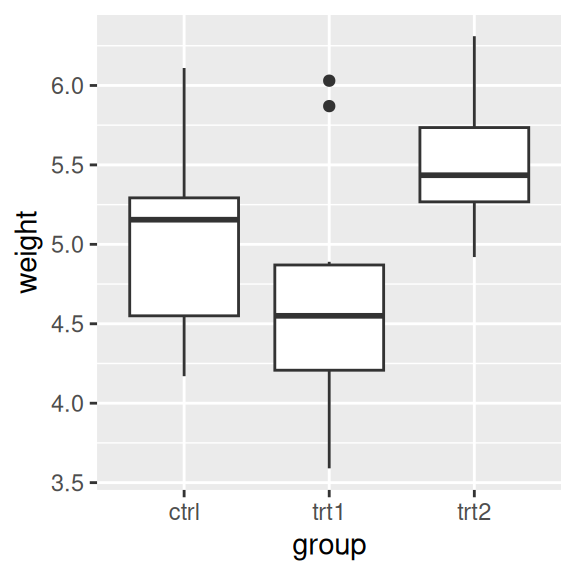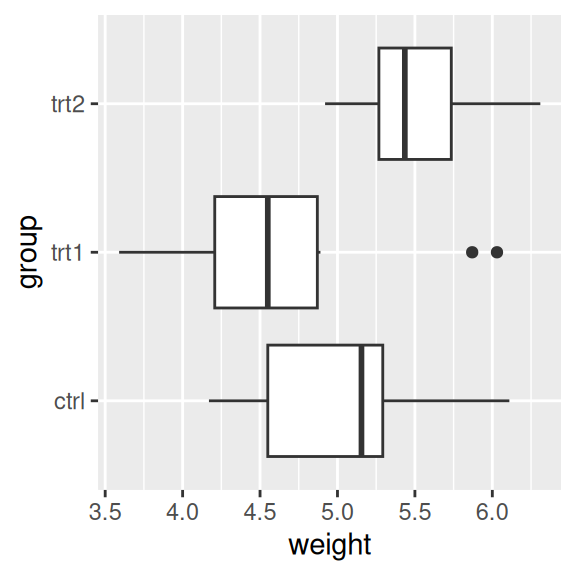Figure 8.1: A box plot with regular axes (left); With swapped axes (right)

### 8.1.3 Discussion

For a scatter plot, it is trivial to change what goes on the vertical axis and what goes on the horizontal axis: just exchange the variables mapped to x and y. But not all the geoms in ggplot treat the x- and y-axes equally. For example, box plots summarize the data along the y-axis, the lines in line graphs move in only one direction along the x-axis, error bars have a single x value and a range of y values, and so on. If you’re using these geoms and want them to behave as though the axes are swapped, `coord_flip()` is what you need.

Sometimes when the axes are swapped, the order of items will be the reverse of what you want. On a graph with standard x- and y-axes, the x items start at the left and go to the right, which corresponds to the normal way of reading, from left to right. When you swap the axes, the items still go from the origin outward, which in this case will be from bottom to top – but this conflicts with the normal way of reading, from top to bottom. Sometimes this is a problem, and sometimes it isn’t. If the x variable is a factor, the order can be reversed by using `scale_x_\$discrete()` with `limits = rev(levels(...))`, as in Figure 8.2:

``````ggplot(PlantGrowth, aes(x = group, y = weight)) +
geom_boxplot() +
coord_flip() +
scale_x_discrete(limits = rev(levels(PlantGrowth\$group)))``````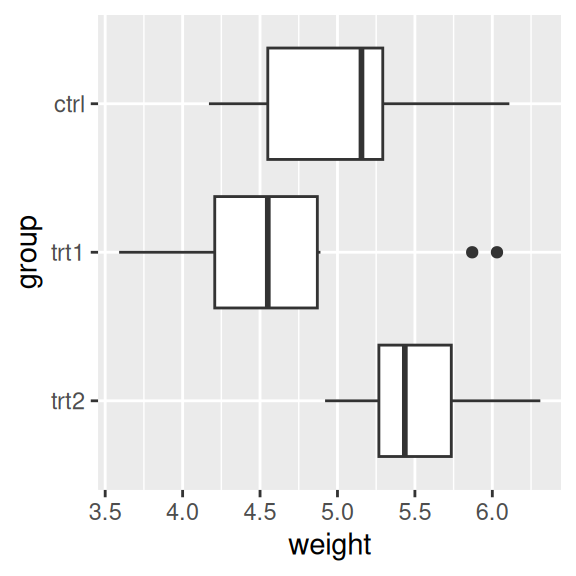Figure 8.2: A box plot with swapped axes and x-axis order reversed

If the variable is continuous, see Recipe 8.3 to reverse the direction.

## 8.2 Setting the Range of a Continuous Axis

### 8.2.1 Problem

You want to set the range (or limits) of an axis.

### 8.2.2 Solution

You can use `xlim()` or `ylim()` to set the minimum and maximum values of a continuous axis. Figure 8.3 shows one graph with the default y limits, and one with manually set y limits:

``````pg_plot <- ggplot(PlantGrowth, aes(x = group, y = weight)) +
geom_boxplot()

# Display the basic graph
pg_plot

pg_plot +
ylim(0, max(PlantGrowth\$weight))``````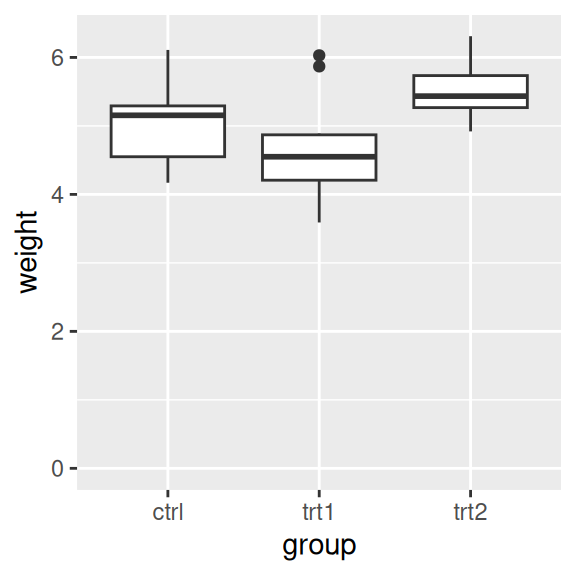Figure 8.3: Box plot with default range (left); With manually set range (right)

The latter example sets the y range from 0 to the maximum value of the `weight` column, though a constant value (like 10) could instead be used as the maximum.

### 8.2.3 Discussion

`ylim()` is shorthand for setting the limits with `scale_y_continuous()`. (The same is true for `xlim()` and `scale_x_continuous()`.) The following are equivalent:

``````ylim(0, 10)
scale_y_continuous(limits = c(0, 10))``````

Sometimes you will need to set other properties of `scale_y_continuous()`, and in these cases using `xlim()` and `scale_y_continuous()` together may result in some unexpected behavior, because only the first of the directives will have an effect. In these two examples, `ylim(0, 10)` should set the y range from 0 to 10, and `scale_y_continuous(breaks=c(0, 5, 10))` should put tick marks at 0, 5, and 10. However, in both cases, only the second directive has any effect:

``````pg_plot +
ylim(0, 10) +
scale_y_continuous(breaks = NULL)

pg_plot +
scale_y_continuous(breaks = NULL) +
ylim(0, 10)``````

To make both changes work, get rid of `ylim()` and set both limits and breaks in `scale_y_continuous()`:

``````pg_plot +
scale_y_continuous(limits = c(0, 10), breaks = NULL)``````

In ggplot, there are two ways of setting the range of the axes. The first way is to modify the scale, and the second is to apply a coordinate transform. When you modify the limits of the x or y scale, any data outside of the limits is removed – that is, the out-of-range data is not only not displayed, it is removed from consideration entirely. (It will also print a warning when this happens.)

With the box plots in these examples, if you restrict the y range so that some of the original data is clipped, the box plot statistics will be computed based on clipped data, and the shape of the box plots will change.

With a coordinate transform, the data is not clipped; in essence, it zooms in or out to the specified range. Figure 8.4 shows the difference between the two methods:

``````pg_plot +
scale_y_continuous(limits = c(5, 6.5))  # Same as using ylim()
#> Warning: Removed 13 rows containing non-finite values (stat_boxplot).

pg_plot +
coord_cartesian(ylim = c(5, 6.5))``````Figure 8.4: Smaller y range using a scale (data has been dropped, so the box plots have changed shape; left); “Zooming in” using a coordinate transform (right)

Finally, it’s also possible to expand the range in one direction, using `expand_limits()` (Figure 8.5). You can’t use this to shrink the range, however:

``````pg_plot +
expand_limits(y = 0)``````Figure 8.5: Box plot on which y range has been expanded to include 0

## 8.3 Reversing a Continuous Axis

### 8.3.1 Problem

You want to reverse the direction of a continuous axis.

### 8.3.2 Solution

Use `scale_y_reverse()` or `scale_x_reverse()` (Figure 8.6). The direction of an axis can also be reversed by specifying the limits in reversed order, with the maximum first, then the minimum:

``````ggplot(PlantGrowth, aes(x = group, y = weight)) +
geom_boxplot() +
scale_y_reverse()

# Similar effect by specifying limits in reversed order
ggplot(PlantGrowth, aes(x = group, y = weight)) +
geom_boxplot() +
ylim(6.5, 3.5)``````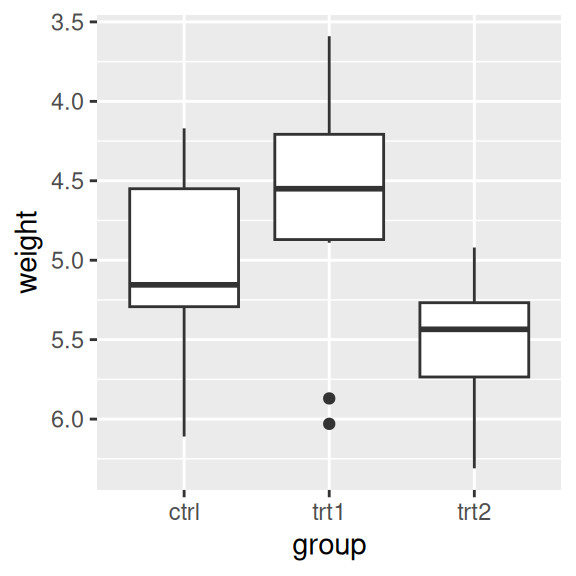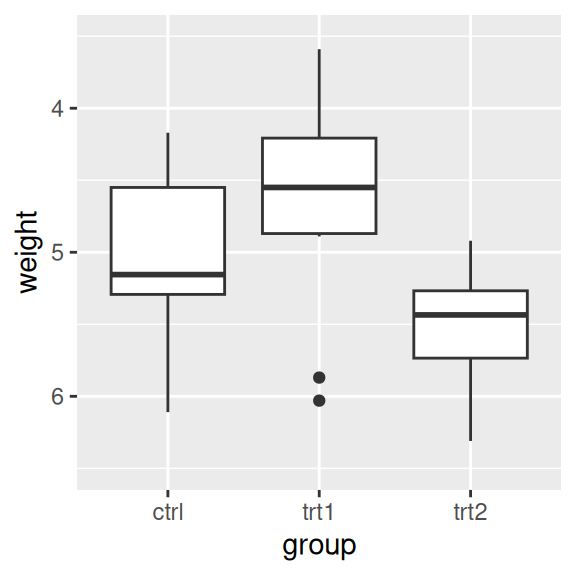Figure 8.6: Box plot with reversed y-axis

### 8.3.3 Discussion

Like `scale_y_continuous()`, `scale_y_reverse()` does not work with `ylim()`. (The same is true for the x-axis properties.) If you want to reverse an axis and set its range, you must do it within the `scale_y_reverse()` statement, by setting the limits in reversed order (Figure 8.7):

``````ggplot(PlantGrowth, aes(x = group, y = weight)) +
geom_boxplot() +
scale_y_reverse(limits = c(8, 0))``````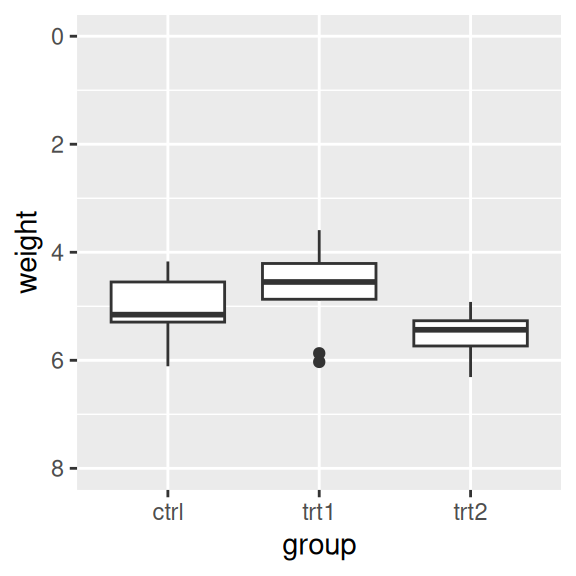Figure 8.7: Box plot with reversed y-axis with manually set limits

To reverse the order of items on a discrete axis, see Recipe 8.4.

## 8.4 Changing the Order of Items on a Categorical Axis

### 8.4.1 Problem

You want to change the order of items on a categorical axis.

### 8.4.2 Solution

For a categorical (or discrete) axis – one with a factor mapped to it – the order of items can be changed by setting limits in `scale_x_discrete()` or `scale_y_discrete()`.

To manually set the order of items on the axis, specify limits with a vector of the levels in the desired order. You can also omit items with this vector, as shown in Figure 8.8, left:

``````pg_plot <- ggplot(PlantGrowth, aes(x = group, y = weight)) +
geom_boxplot()

pg_plot +
scale_x_discrete(limits = c("trt1", "ctrl", "trt2"))``````

### 8.4.3 Discussion

You can also use this method to display a subset of the items on the axis. This will show only `ctrl` and `trt1` (Figure 8.8, right). Note that because data is removed, it will emit a warning when you do this.

``````pg_plot +
scale_x_discrete(limits = c("ctrl", "trt1"))
#> Warning: Removed 10 rows containing missing values (stat_boxplot).``````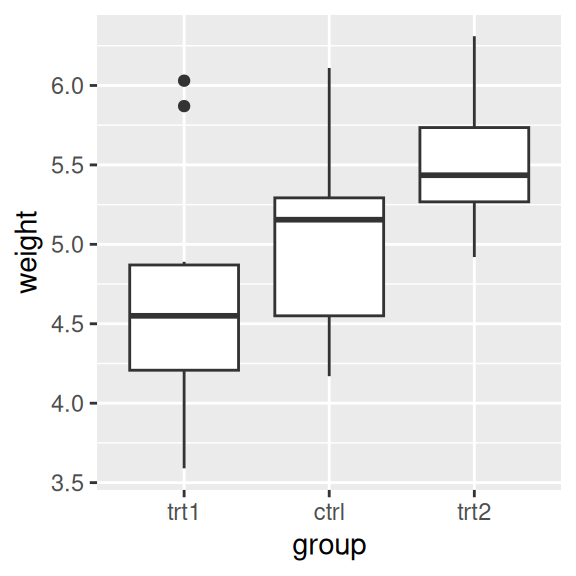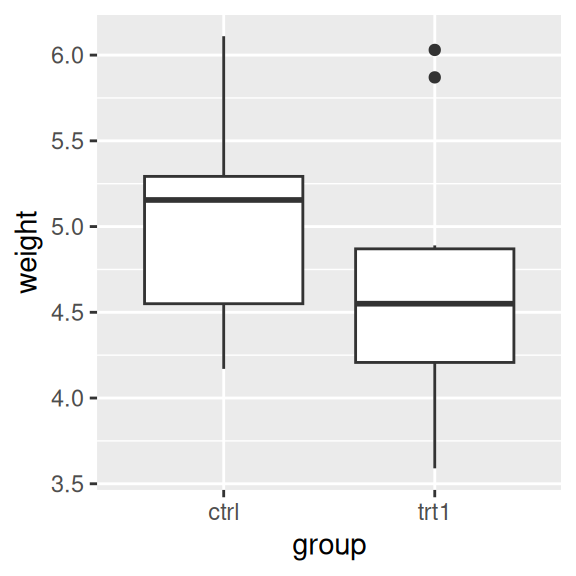Figure 8.8: Box plot with manually specified items on the x-axis (left); With only two items (right)

To reverse the order, set `limits = rev(levels(...))`, and put the factor inside. This will reverse the order of the `PlantGrowth\$group` factor, as shown in Figure 8.9:

``````pg_plot +
scale_x_discrete(limits = rev(levels(PlantGrowth\$group)))``````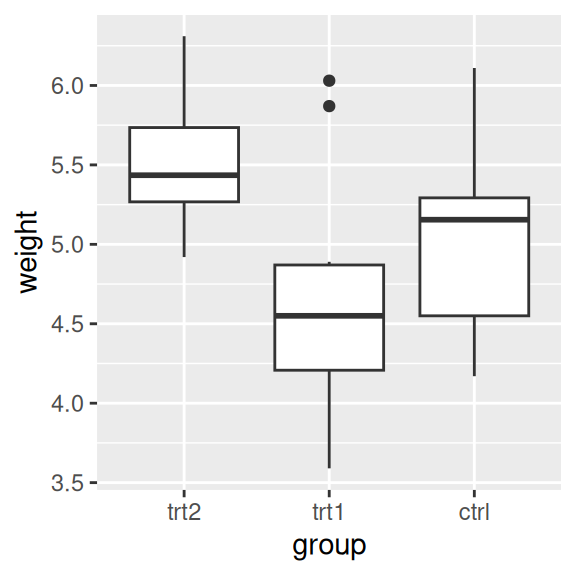Figure 8.9: Box plot with order reversed on the x-axis

To reorder factor levels based on data values from another column, see Recipe 15.9.

## 8.5 Setting the Scaling Ratio of the X- and Y-Axes

### 8.5.1 Problem

You want to set the ratio at which the x- and y-axes are scaled.

### 8.5.2 Solution

Use `coord_fixed()`. This will result in a 1:1 scaling between the x- and y-axes, as shown in Figure 8.10:

``````library(gcookbook)  # Load gcookbook for the marathon data set

m_plot <- ggplot(marathon, aes(x = Half,y = Full)) +
geom_point()

m_plot +
coord_fixed()``````

### 8.5.3 Discussion

The marathon data set contains runners’ marathon and half-marathon times. In this case it might be useful to force the x- and y-axes to have the same scaling.

It’s also helpful to set the tick spacing to be the same, by setting breaks in `scale_y_continuous()` and `scale_x_continuous()` (also in Figure 8.10):

``````m_plot +
coord_fixed() +
scale_y_continuous(breaks = seq(0, 420, 30)) +
scale_x_continuous(breaks = seq(0, 420, 30))``````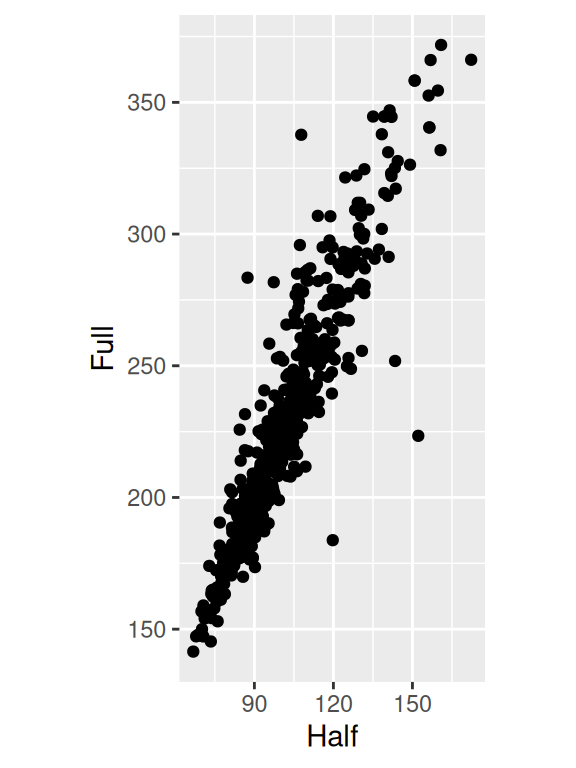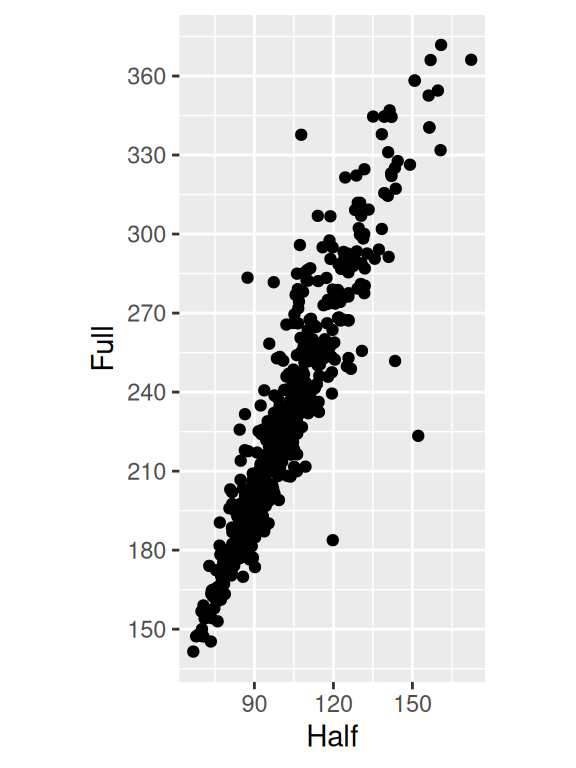Figure 8.10: Scatter plot with equal scaling of axes (left); With tick marks at specified positions (right)

If, instead of an equal ratio, you want some other fixed ratio between the axes, set the ratio parameter. With the marathon data, we might want the axis with half-marathon times stretched out to twice that of the axis with the marathon times (Figure 8.11). We’ll also add tick marks twice as often on the x-axis:

``````m_plot +
coord_fixed(ratio = 1/2) +
scale_y_continuous(breaks = seq(0, 420, 30)) +
scale_x_continuous(breaks = seq(0, 420, 15))``````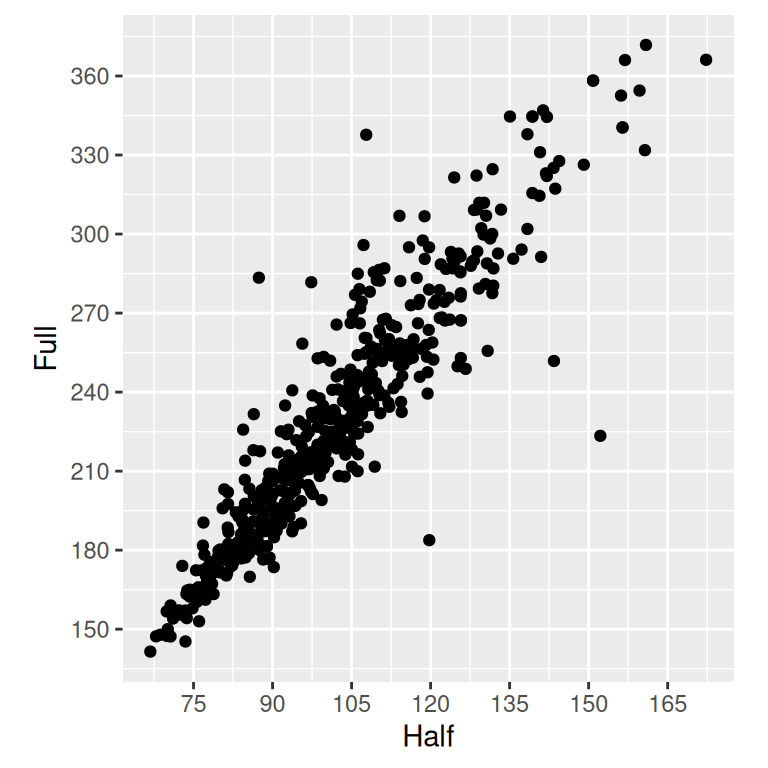Figure 8.11: Scatter plot with a 1/2 scaling ratio for the axes

## 8.6 Setting the Positions of Tick Marks

### 8.6.1 Problem

You want to set where the tick marks appear on the axis.

### 8.6.2 Solution

Usually ggplot does a good job of deciding where to put the tick marks, but if you want to change them, set `breaks` in the scale (Figure 8.12):

``````ggplot(PlantGrowth, aes(x = group, y = weight)) +
geom_boxplot()

ggplot(PlantGrowth, aes(x = group, y = weight)) +
geom_boxplot() +
scale_y_continuous(breaks = c(4, 4.25, 4.5, 5, 6, 8))``````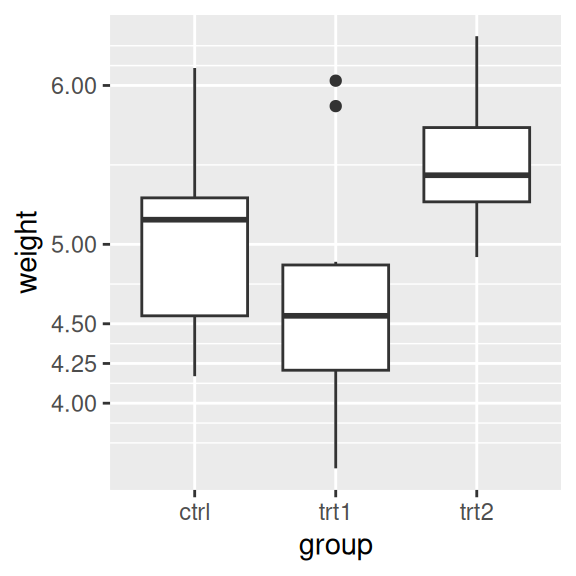Figure 8.12: Box plot with automatic tick marks (left); With manually set tick marks (right)

### 8.6.3 Discussion

The location of the tick marks defines where major grid lines are drawn. If the axis represents a continuous variable, minor grid lines, which are fainter and unlabeled, will by default be drawn halfway between each major grid line.

You can also use the `seq()` function or the `:` operator to generate vectors for tick marks:

``````seq(4, 7, by = .5)
#>  4.0 4.5 5.0 5.5 6.0 6.5 7.0
5:10
#>   5  6  7  8  9 10``````

If the axis is discrete instead of continuous, then there is by default a tick mark for each item. For discrete axes, you can change the order of items or remove them by specifying the limits (see Recipe 8.4). Setting breaks will change which of the levels are labeled, but will not remove them or change their order. Figure 8.13 shows what happens when you set limits and breaks (the warning is because we’re using only two of the three levels for `group` and therefore are dropping some rows):

``````# Set both breaks and labels for a discrete axis
ggplot(PlantGrowth, aes(x = group, y = weight)) +
geom_boxplot() +
scale_x_discrete(limits = c("trt2", "ctrl"), breaks = "ctrl")
#> Warning: Removed 10 rows containing missing values (stat_boxplot).``````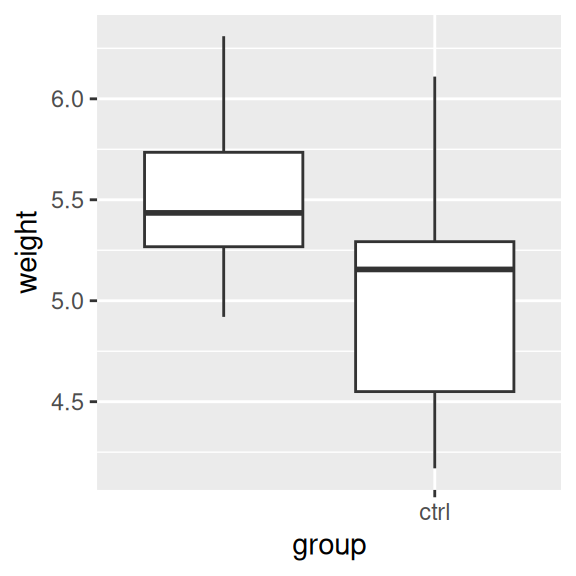Figure 8.13: For a discrete axis, setting limits reorders and removes items, and setting breaks controls which items have labels

To remove the tick marks and labels (but not the data) from thegraph, see Recipe 8.7.

## 8.7 Removing Tick Marks and Labels

### 8.7.1 Problem

You want to remove tick marks and labels.

### 8.7.2 Solution

To remove just the tick labels, as in Figure 8.14 (left), use `theme(axis.text.y = element_blank()`) (or do the same for `axis.text.x`). This will work for both continuous and categorical axes:

``````pg_plot <- ggplot(PlantGrowth, aes(x = group, y = weight)) +
geom_boxplot()

pg_plot +
theme(axis.text.y = element_blank())``````

To remove the tick marks, use `theme(axis.ticks=element_blank())`. This will remove the tick marks on both axes. (It’s not possible to hide the tick marks on just one axis.) In this example, we’ll hide all tick marks as well as the y tick labels (Figure 8.14, center):

``````pg_plot +
theme(axis.ticks = element_blank(), axis.text.y = element_blank())``````

To remove the tick marks, the labels, and the grid lines, set breaks to `NULL` (Figure 8.14, right):

``````pg_plot +
scale_y_continuous(breaks = NULL)``````
(ref:cap-FIG-AXES-SET-TICKS-NONE) No tick labels on y-axis (left); No tick marks and no tick labels on y-axis (middle); With `breaks=NULL` (right)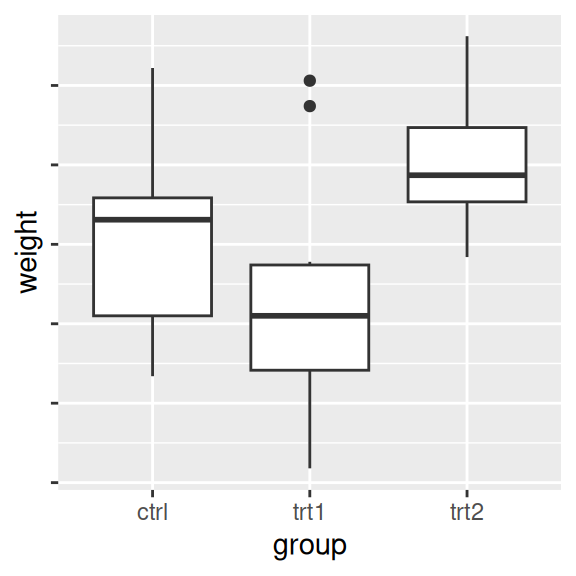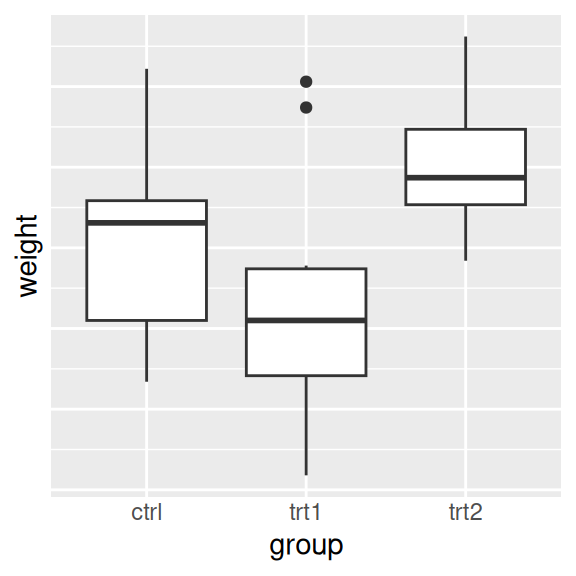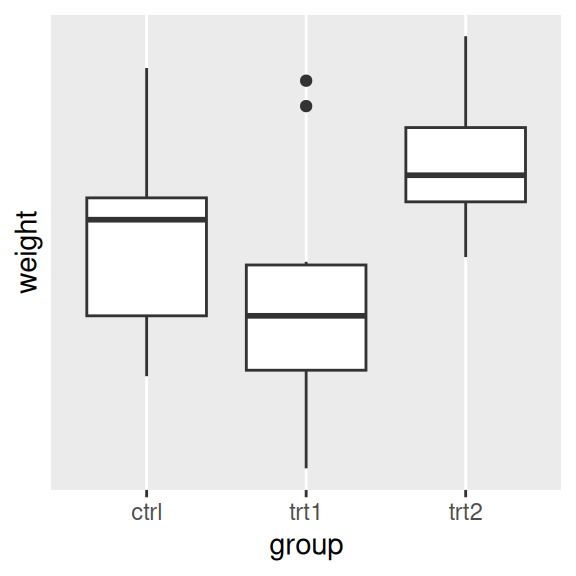Figure 8.14: (ref:cap-FIG-AXES-SET-TICKS-NONE)

This will work for continuous axes only; if you remove items from a categorical axis using limits, as in Recipe 8.4, the data with that value won’t be shown at all.

### 8.7.3 Discussion

There are actually three related items that can be controlled: tick labels, tick marks, and the grid lines. For continuous axes, `ggplot()` normally places a tick label, tick mark, and major grid line at each value of breaks. For categorical axes, these things go at each value of limits.

The tick labels on each axis can be controlled independently. However, the tick marks and grid lines must be controlled all together.

## 8.8 Changing the Text of Tick Labels

### 8.8.1 Problem

You want to change the text of tick labels.

### 8.8.2 Solution

Consider the scatter plot in Figure 8.15, where height is reported in inches:

``````library(gcookbook)  # Load gcookbook for the heightweight data set

hw_plot <- ggplot(heightweight, aes(x = ageYear, y = heightIn)) +
geom_point()

hw_plot``````

To set arbitrary labels, as in Figure 8.15 (right), pass values to breaks and labels in the scale. One of the labels has a newline (`\n`) character, which tells ggplot to put a line break there:

``````hw_plot +
scale_y_continuous(
breaks = c(50, 56, 60, 66, 72),
labels = c("Tiny", "Really\nshort", "Short", "Medium", "Tallish")
)``````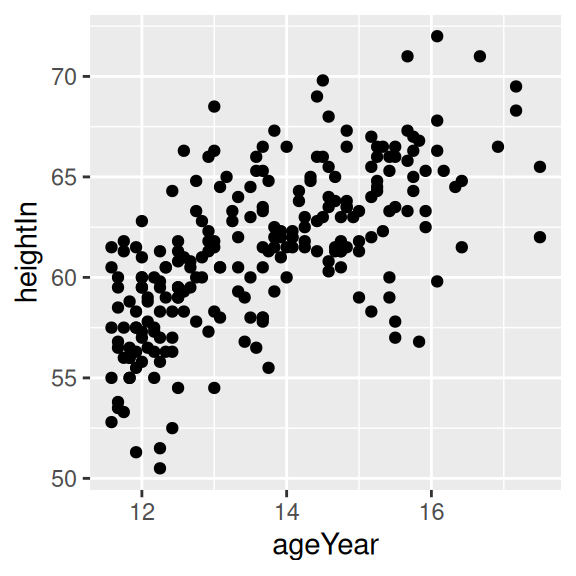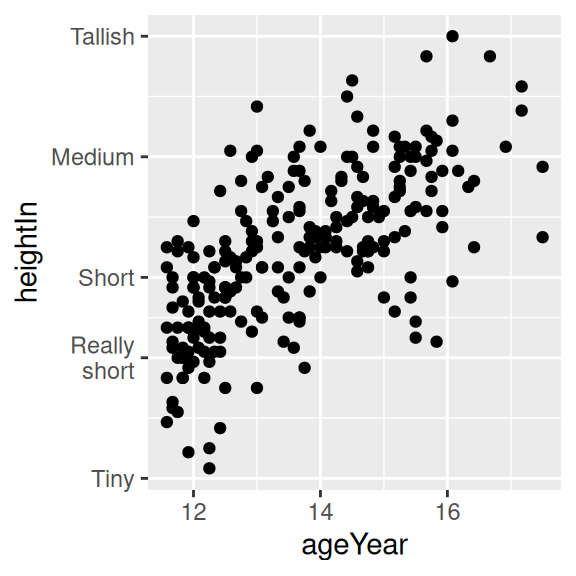Figure 8.15: Scatter plot with automatic tick labels (left); With manually specified labels on the y-axis (right)

### 8.8.3 Discussion

Instead of setting completely arbitrary labels, it is more common to have your data stored in one format, while wanting the labels to be displayed in another. We might, for example, want heights to be displayed in feet and inches (like 5’6“) instead of just inches. To do this, we can define a formatter function, which takes in a value and returns the corresponding string. For example, this function will convert inches to feet and inches:

``````footinch_formatter <- function(x) {
foot <- floor(x/12)
inch <- x %% 12
return(paste(foot, "'", inch, "\"", sep = ""))
}``````

Here’s what it returns for values 56–64 (the backslashes are there as escape characters, to distinguish the quotes in a string from the quotes that delimit a string):

``````footinch_formatter(56:64)
#>  "4'8\""  "4'9\""  "4'10\"" "4'11\"" "5'0\""  "5'1\""  "5'2\""  "5'3\""
#>  "5'4\""``````

Now we can pass our function to the scale, using the labels parameter (Figure 8.16, left):

``````hw_plot +
scale_y_continuous(labels = footinch_formatter)``````

Here, the automatic tick marks were placed every five inches, but that looks a little off for this data. We can instead have ggplot set tick marks every four inches, by specifying breaks (Figure 8.16, right):

``````hw_plot +
scale_y_continuous(breaks = seq(48, 72, 4), labels = footinch_formatter)``````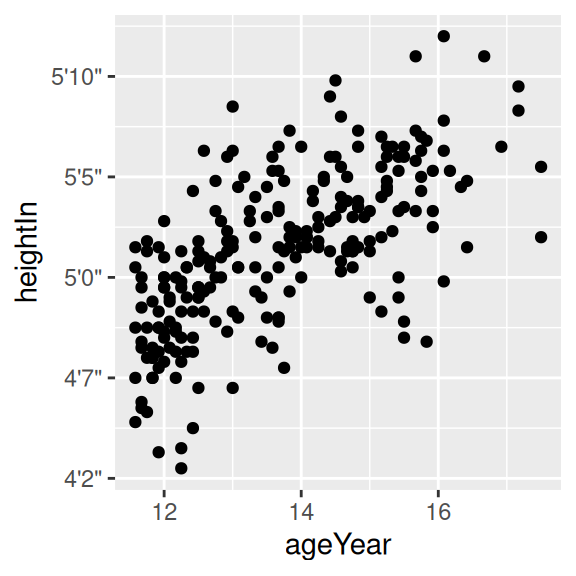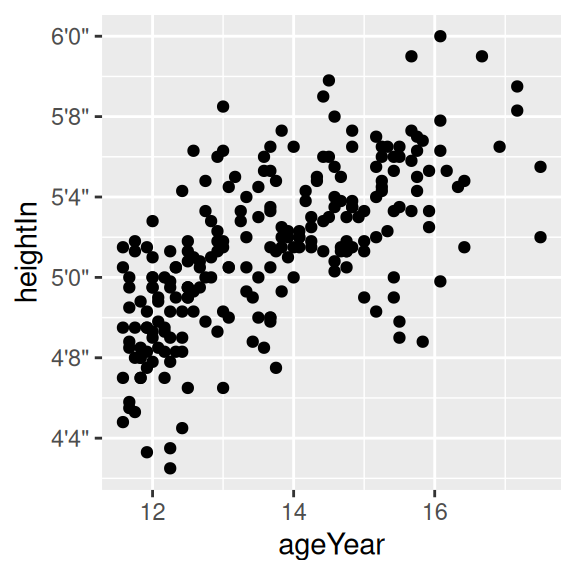Figure 8.16: Scatter plot with a formatter function (left); With manually specified breaks on the y-axis (right)

Another common task is to convert time measurements to HH:MM:SS format, or something similar. This function will take numeric minutes and convert them to this format, rounding to the nearest second (it can be customized for your particular needs):

``````timeHMS_formatter <- function(x) {
h <- floor(x/60)
m <- floor(x %% 60)
s <- round(60*(x %% 1))                   # Round to nearest second
lab <- sprintf("%02d:%02d:%02d", h, m, s) # Format the strings as HH:MM:SS
lab <- gsub("^00:", "", lab)              # Remove leading 00: if present
lab <- gsub("^0", "", lab)                # Remove leading 0 if present
return(lab)
}``````

Running it on some sample numbers yields:

``````timeHMS_formatter(c(.33, 50, 51.25, 59.32, 60, 60.1, 130.23))
#>  "0:20"    "50:00"   "51:15"   "59:19"   "1:00:00" "1:00:06" "2:10:14"``````

The scales package, which is installed with ggplot2, comes with some built-in formatting functions:

• `comma()` adds commasto numbers, in the thousand, million, billion, etc. places.
• `dollar()` adds a dollar sign and rounds to the nearest cent.
• `percent()` multiplies by 100, rounds to the nearest integer, and adds a percent sign.
• `scientific()` gives numbers in scientific notation, like `3.30e+05`, for large and small numbers.

If you want to use these functions, you must first load the scales package, with `library(scales)`.

## 8.9 Changing the Appearance of Tick Labels

### 8.9.1 Problem

You want to change the appearance of tick labels.

### 8.9.2 Solution

In Figure 8.17 (left), we’ve manually set the labels to be long-long enough that they overlap:

``````pg_plot <- ggplot(PlantGrowth, aes(x = group, y = weight)) +
geom_boxplot() +
scale_x_discrete(
breaks = c("ctrl", "trt1", "trt2"),
labels = c("Control", "Treatment 1", "Treatment 2")
)

pg_plot``````

To rotate the text 90 degrees counterclockwise (Figure 8.17, middle), use:

``````pg_plot +
theme(axis.text.x = element_text(angle = 90, hjust = 1, vjust = .5))``````

Rotating the text 30 degrees (Figure 8.17, right) uses less vertical space and makes the labels easier to read without tilting your head:

``````pg_plot +
theme(axis.text.x = element_text(angle = 30, hjust = 1, vjust = 1))``````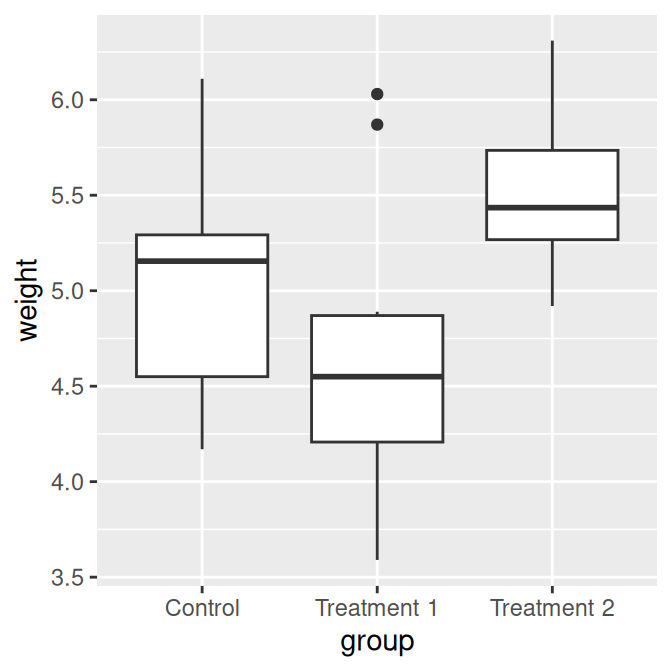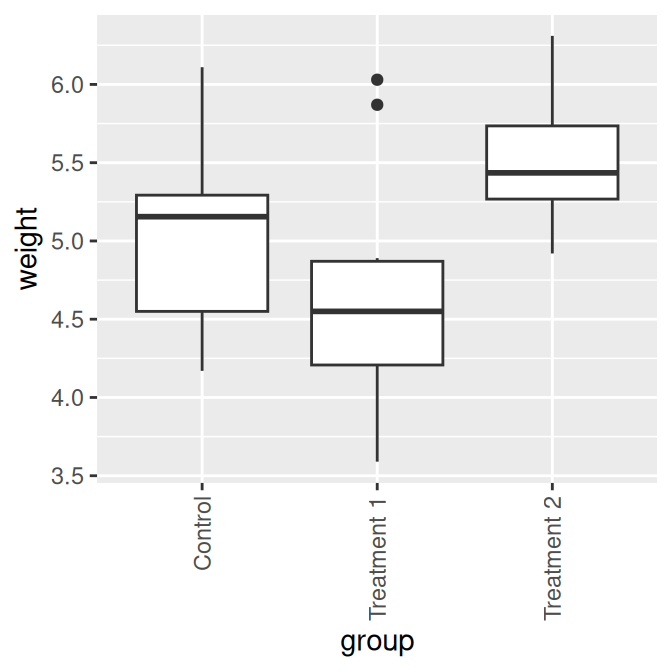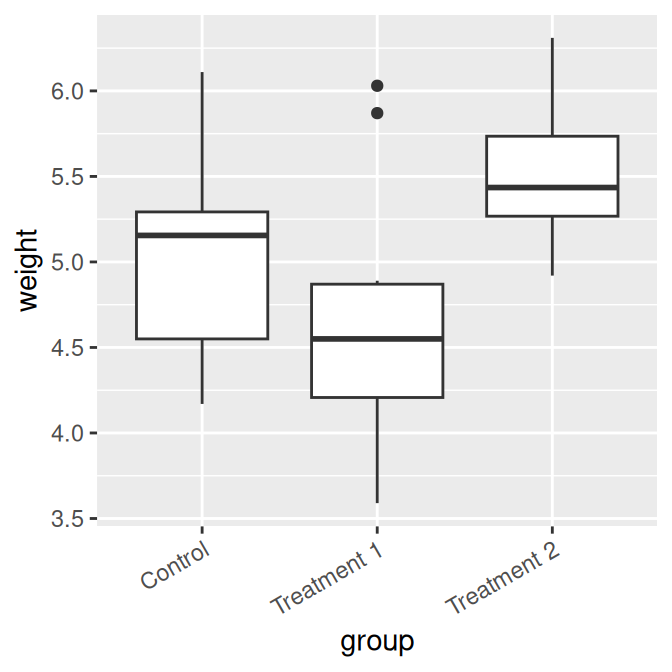Figure 8.17: X-axis tick labels rotated 0 (left), 90 (middle), and 30 degrees (right)

The `hjust` and `vjust` settings specify the horizontal alignment (left/center/right) and vertical alignment (top/middle/bottom).

### 8.9.3 Discussion

Besides rotation, other text properties, such as size, style (bold/italic/normal), and the font family (such as Times or Helvetica) can be set with `element_text()`, as shown in Figure 8.18:

``````pg_plot +
theme(
axis.text.x = element_text(family = "Times", face = "italic",
colour = "darkred", size = rel(0.9))
)``````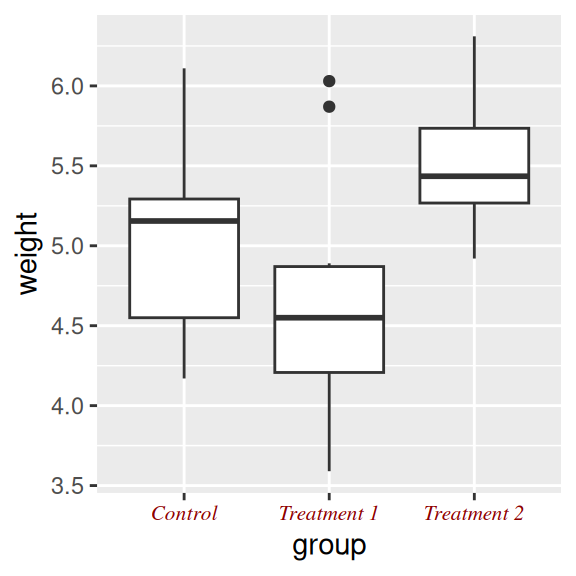Figure 8.18: X-axis tick labels with manually specified appearance

In this example, the size is set to `rel(0.9)`, which means that it is 0.9 times the size of the base font size for the theme.

These commands control the appearance of only the tick labels, on only one axis. They don’t affect the other axis, the axis label, the overall title, or the legend. To control all of these at once, you can use the theming system, as discussed in Recipe 9.3.

See Recipe 9.2 for more about controlling the appearance of the text.

## 8.10 Changing the Text of Axis Labels

### 8.10.1 Problem

You want to change the text of axis labels.

### 8.10.2 Solution

Use `xlab()` or `ylab()` to change the text of the axis labels (Figure 8.19):

``````library(gcookbook)  # Load gcookbook for the heightweight data set

hw_plot <- ggplot(heightweight, aes(x = ageYear, y = heightIn, colour = sex)) +
geom_point()

# With default axis labels
hw_plot

# Set the axis labels
hw_plot +
xlab("Age in years") +
ylab("Height in inches")``````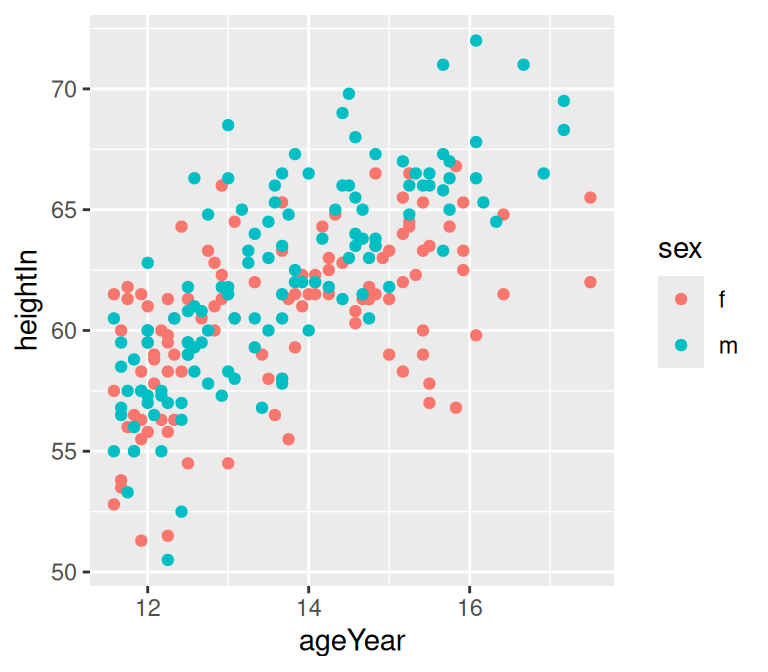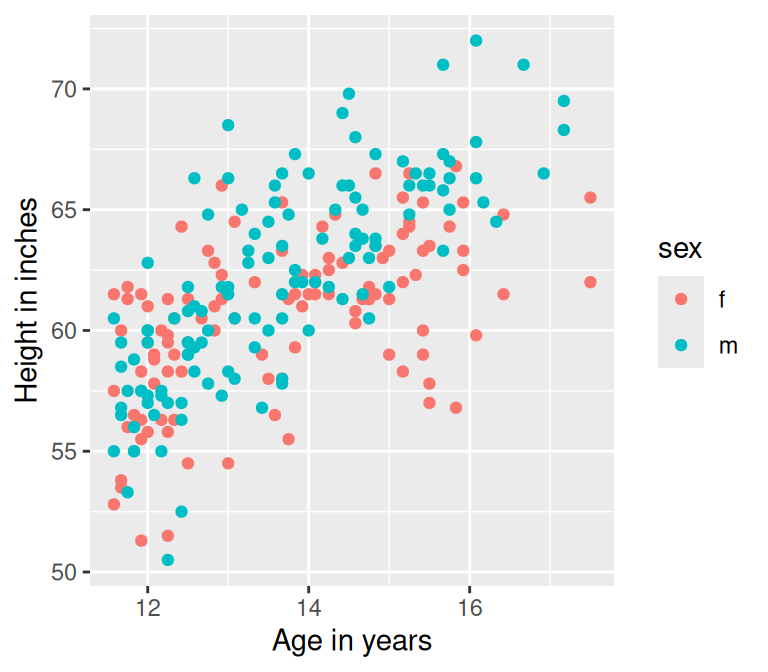Figure 8.19: Scatter plot with the default axis labels (left); Manually specified labels for the x- and y-axes (right)

### 8.10.3 Discussion

By default the graphs will just use the column names from the data frame as axis labels. This might be fine for exploring data, but for presenting it, you may want more descriptive axis labels.

Instead of `xlab()` and `ylab()`, you can use `labs()`:

``````hw_plot +
labs(x = "Age in years", y = "Height in inches")``````

Another way of setting the axis labels is in the scale specification, like this:

``````hw_plot +
scale_x_continuous(name = "Age in years")``````

This may look a bit awkward, but it can be useful if you’re also setting other properties of the scale, such as the tick mark placement, range, and so on.

This also applies, of course, to other axis scales, such as `scale_y_continuous()`, `scale_x_discrete()`, and so on.

You can also add line breaks with `\n`, as shown in Figure 8.20:

``````hw_plot +
scale_x_continuous(name = "Age\n(years)")``````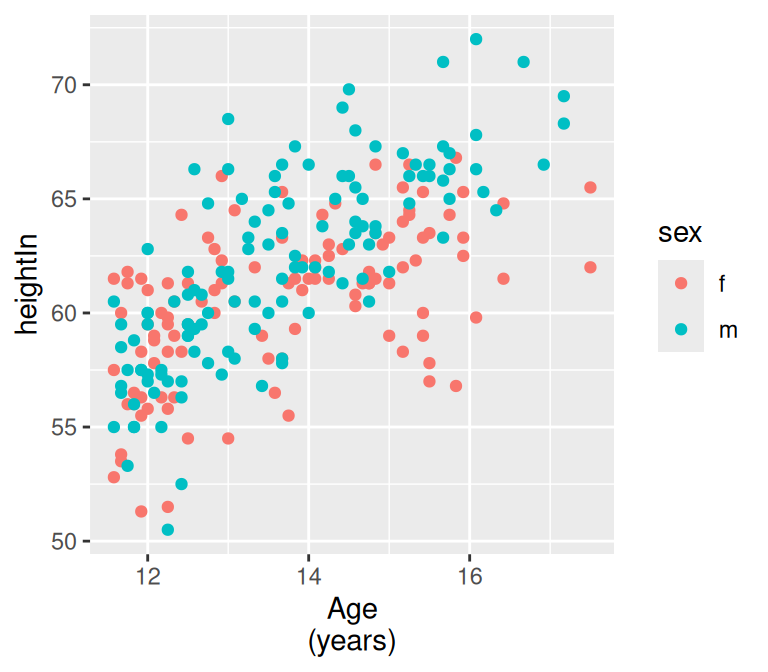Figure 8.20: X-axis label with a line break

## 8.11 Removing Axis Labels

### 8.11.1 Problem

You want to remove the label on an axis.

### 8.11.2 Solution

For the x-axis label, use `xlab(NULL)`. For the y-axis label, use `ylab(NULL)`.

We’ll hide the x-axis in this example (Figure 8.21):

``````pg_plot <- ggplot(PlantGrowth, aes(x = group, y = weight)) +
geom_boxplot()

pg_plot +
xlab(NULL)``````

### 8.11.3 Discussion

Sometimes axis labels are redundant or obvious from the context, and don’t need to be displayed. In the example here, the x-axis represents group, but this should be obvious from the context. Similarly, if the y tick labels had kg or some other unit in each label, the axis label “weight” would be unnecessary.

Another way to remove the axis label is to set it to an empty string. However, if you do it this way, the resulting graph will still have space reserved for the text, as shown in the graph on the right in Figure 8.21:

``````pg_plot +
xlab("")``````Figure 8.21: X-axis label with `NULL` (left); With the label set to `""` (right)

When you use `theme()` to set `axis.title.x = element_blank()`, the name of the x or y scale is unchanged, but the text is not displayed and no space is reserved for it. When you set the label to `""`, the name of the scale is changed and the (empty) text does display.

## 8.12 Changing the Appearance of Axis Labels

### 8.12.1 Problem

You want to change the appearance of axis labels.

### 8.12.2 Solution

To change the appearance of the x-axis label (Figure 8.22), use `axis.title.x`:

``````library(gcookbook)  # Load gcookbook for the heightweight data set

hw_plot <- ggplot(heightweight, aes(x = ageYear, y = heightIn)) +
geom_point()

hw_plot +
theme(axis.title.x = element_text(face = "italic", colour = "darkred", size = 14))``````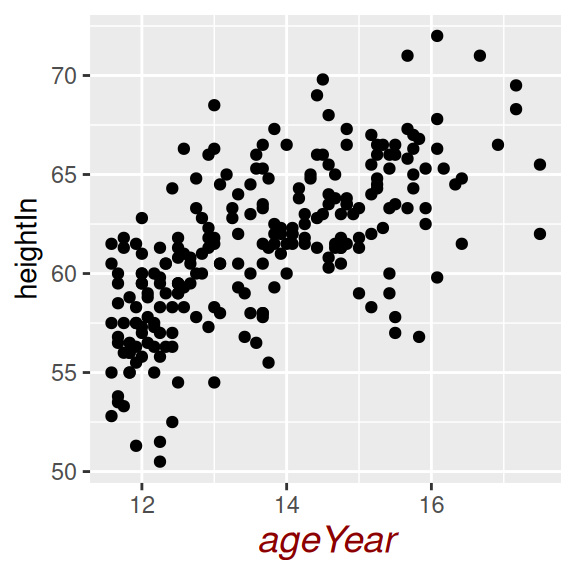Figure 8.22: X-axis label with customized appearance

### 8.12.3 Discussion

For the y-axis label, it might also be useful to display the text unrotated, as shown in Figure 8.23 (left). The `\n` in the label represents a newline character:

``````hw_plot +
ylab("Height\n(inches)") +
theme(axis.title.y = element_text(angle = 0, face = "italic", size = 14))``````

When you call `element_text()`, the default `angle` is 0, so if you set `axis.title.y` but don’t specify the `angle`, it will show in this orientation, with the top of the text pointing up. If you change any other properties of `axis.title.y` and want it to be displayed in its usual orientation, rotated 90 degrees, you must manually specify the `angle` (Figure 8.23, right):

``````hw_plot +
ylab("Height\n(inches)") +
theme(axis.title.y = element_text(
angle = 90,
face = "italic",
colour = "darkred",
size = 14)
)``````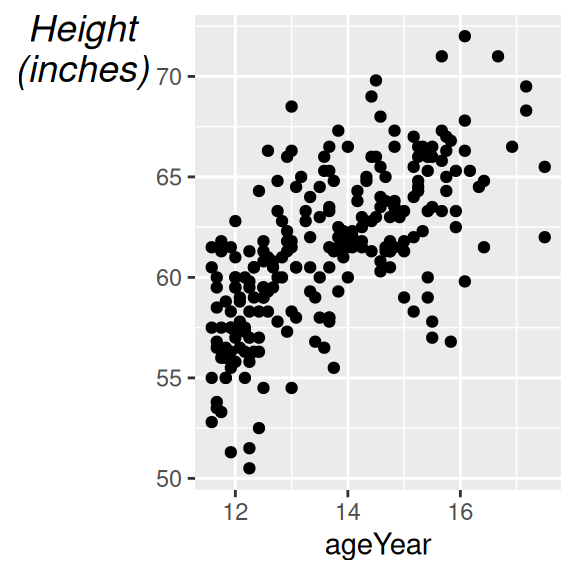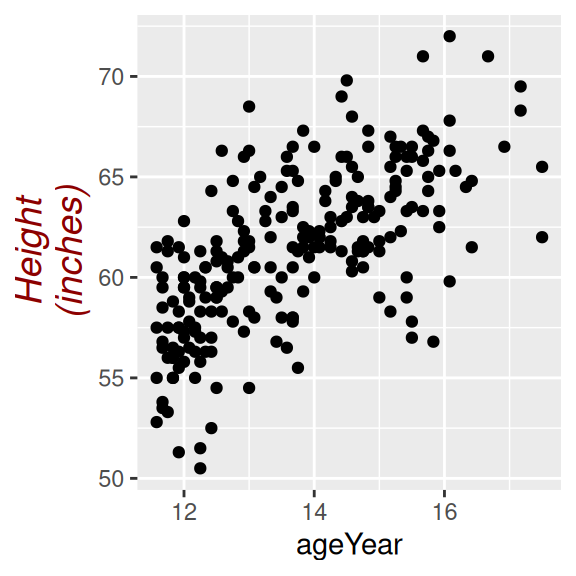Figure 8.23: Y-axis label with angle = 0 (left); With angle = 90 (right)

See Recipe 9.2 for more about controlling the appearance of the text.

## 8.13 Showing Lines Along the Axes

### 8.13.1 Problem

You want to display lines along the x- and y-axes, but not on the other sides of the graph.

### 8.13.2 Solution

Using themes, use `axis.line` (Figure 8.24):

``````library(gcookbook)  # Load gcookbook for the heightweight data set

hw_plot <- ggplot(heightweight, aes(x = ageYear, y = heightIn)) +
geom_point()

hw_plot +
theme(axis.line = element_line(colour = "black"))``````

### 8.13.3 Discussion

If you are starting with a theme that has a border around the plotting area, like theme_bw(), you will also need to unset panel.border (Figure 8.24, right):

``````hw_plot +
theme_bw() +
theme(panel.border = element_blank(), axis.line = element_line(colour = "black"))``````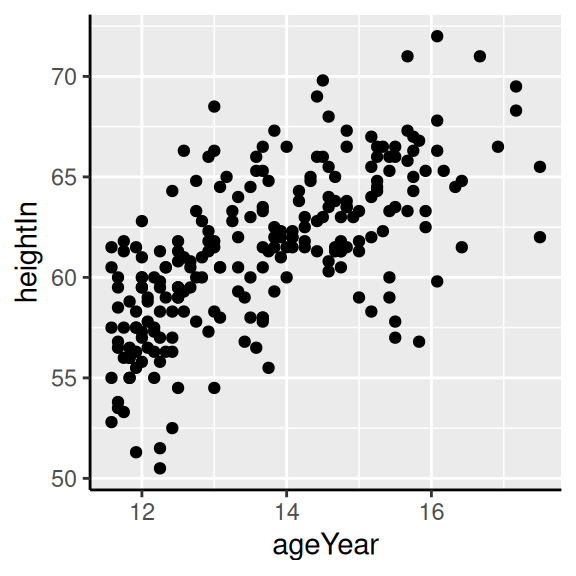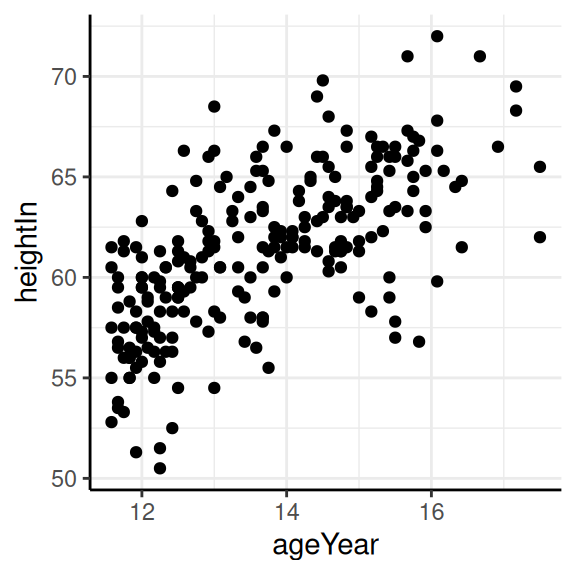Figure 8.24: Scatter plot with axis lines (left); With `theme_bw()`, `panel.border` must also be made blank (right)

If the lines are thick, the ends will only partially overlap (Figure 8.25, left). To make them fully overlap (Figure 8.25, right), set `lineend = "square"`:

``````# With thick lines, only half overlaps
hw_plot +
theme_bw() +
theme(
panel.border = element_blank(),
axis.line = element_line(colour = "black", size = 4)
)

# Full overlap
hw_plot +
theme_bw() +
theme(
panel.border = element_blank(),
axis.line = element_line(colour = "black", size = 4, lineend = "square")
)``````Figure 8.25: With thick lines, the ends don’t fully overlap (left); Full overlap with `lineend="square"` (right)

For more information about how the theming system works, see Recipe 9.3.

## 8.14 Using a Logarithmic Axis

### 8.14.1 Problem

You want to use a logarithmic axis for a graph.

### 8.14.2 Solution

Use `scale_x_log10()` and/or `scale_y_log10()` (Figure 8.26):

``````library(MASS) # Load MASS for the Animals data set

# Create the base plot
animals_plot <- ggplot(Animals, aes(x = body, y = brain, label = rownames(Animals))) +
geom_text(size = 3)

animals_plot

# With logarithmic x and y scales
animals_plot +
scale_x_log10() +
scale_y_log10()``````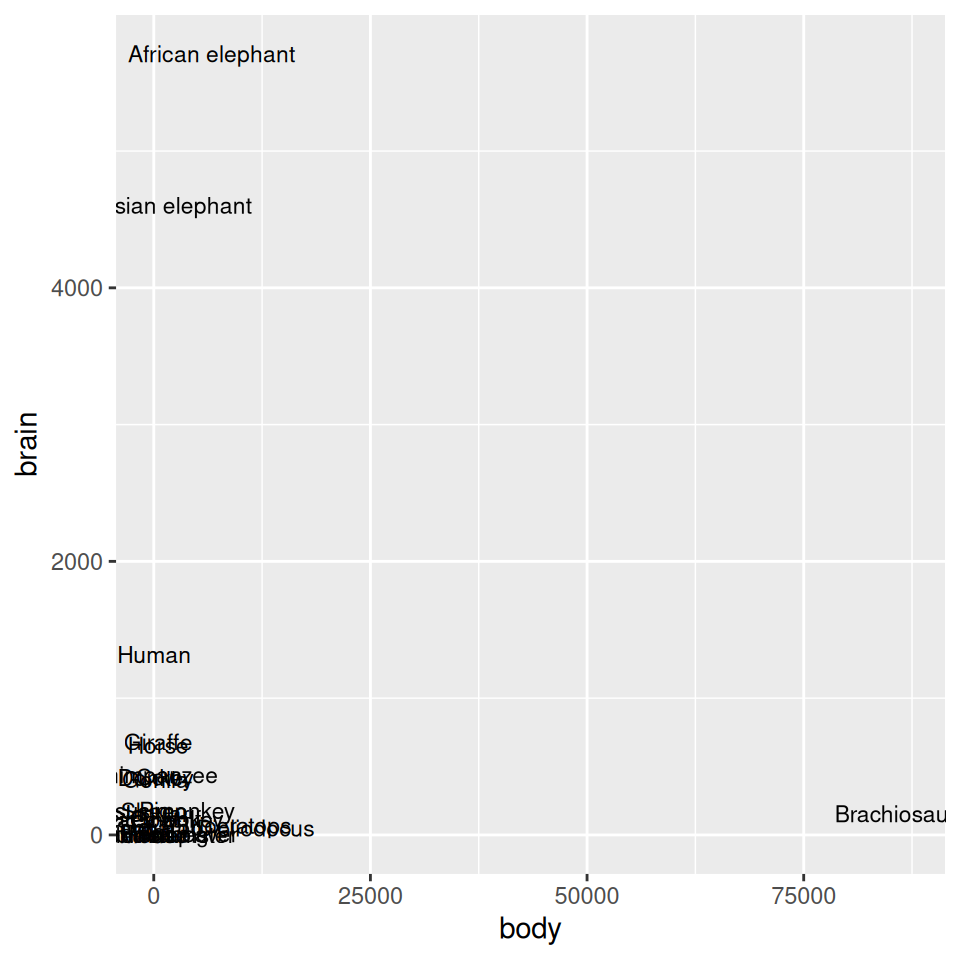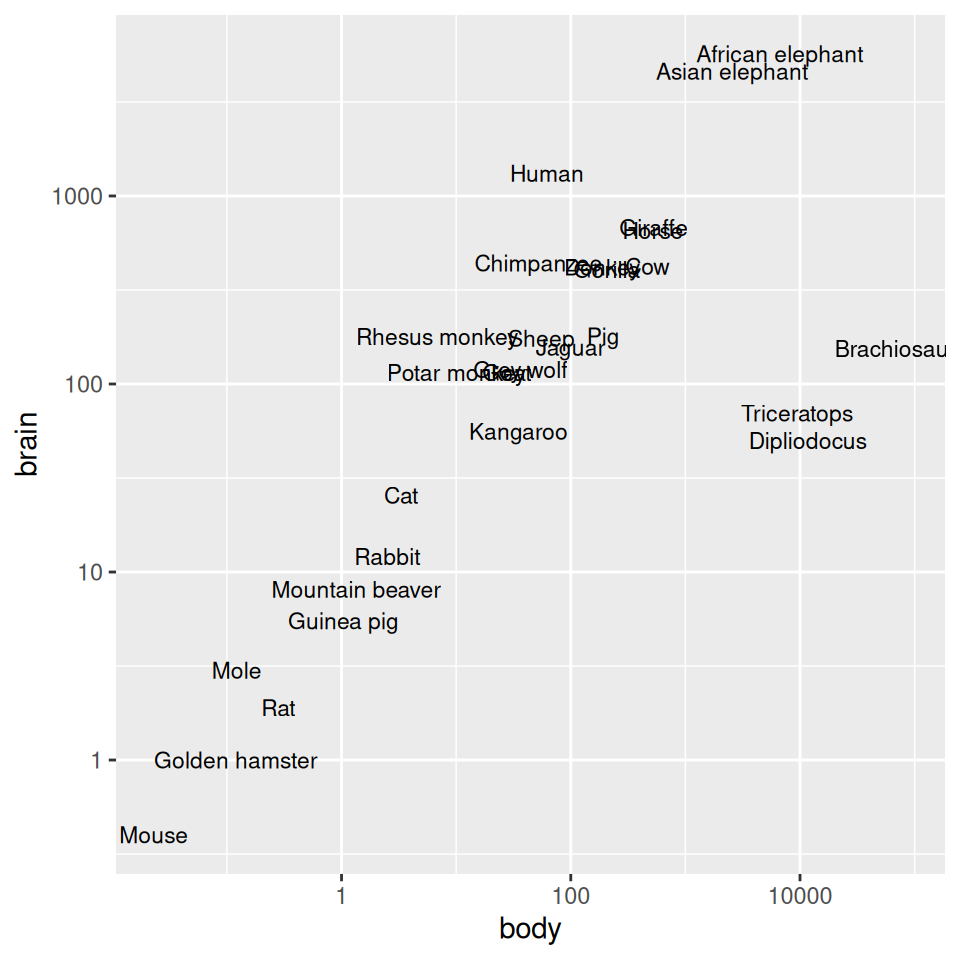Figure 8.26: Exponentially distributed data with linear-scaled axes (left); With logarithmic axes (right)

### 8.14.3 Discussion

With a log axis, a given visual distance represents a constant proportional change; for example, each centimeter on the y-axis might represent a multiplication of the quantity by 10. In contrast, with a linear axis, a given visual distance represents a constant quantity change; each centimeter might represent adding 10 to the quantity.

Some data sets are exponentially distributed on the x-axis, and others on the y-axis (or both). For example, the `Animals` data set from the MASS package contains data on the average brain mass (in g) and body mass (in kg) of various mammals, with a few dinosaurs thrown in for comparison:

``````Animals
#>                      body brain
#> Mountain beaver     1.350   8.1
#> Cow               465.000 423.0
#> Grey wolf          36.330 119.5
#>  ...<22 more rows>...
#> Brachiosaurus   87000.000 154.5
#> Mole                0.122   3.0
#> Pig               192.000 180.0``````

As shown in Figure 8.26, we can make a scatter plot to visualize the relationship between brain and body mass. With the default linearly scaled axes, it’s hard to make much sense of this graph. Because of a few very large animals, the rest of the animals get squished into the lower-left corner-a mouse barely looks different from a triceratops! This is a case where the data is distributed exponentially on both axes.

ggplot will try to make good decisions about where to place the tick marks, but if you don’t like them, you can change them by specifying `breaks` and, optionally, `labels`. In the example here, the automatically generated tick marks are spaced farther apart than is ideal. For the y-axis tick marks, we can get a vector of every power of 10 from 100 to 103 like this:

``````10^(0:3)
#>     1   10  100 1000``````

The x-axis tick marks work the same way, but because the range is large, R decides to format the output with scientific notation:

``````10^(-1:5)
#>  1e-01 1e+00 1e+01 1e+02 1e+03 1e+04 1e+05``````

And then we can use those values as the breaks, as in Figure 8.27 (left):

``````animals_plot +
scale_x_log10(breaks = 10^(-1:5)) +
scale_y_log10(breaks = 10^(0:3))``````

To instead use exponential notation for the break labels (Figure 8.27, right), use the trans_format() function, from the scales package:

``````library(scales)
animals_plot +
scale_x_log10(breaks = 10^(-1:5), labels = trans_format("log10", math_format(10^.x))) +
scale_y_log10(breaks = 10^(0:3), labels = trans_format("log10", math_format(10^.x)))``````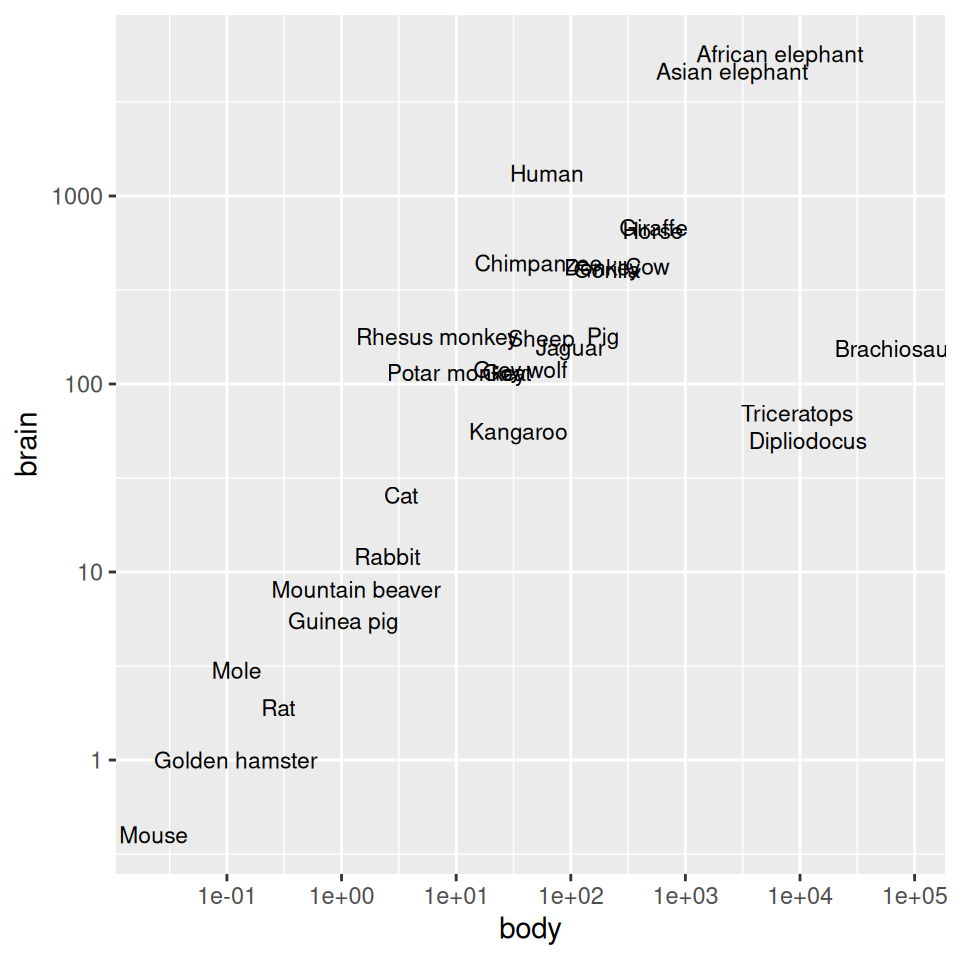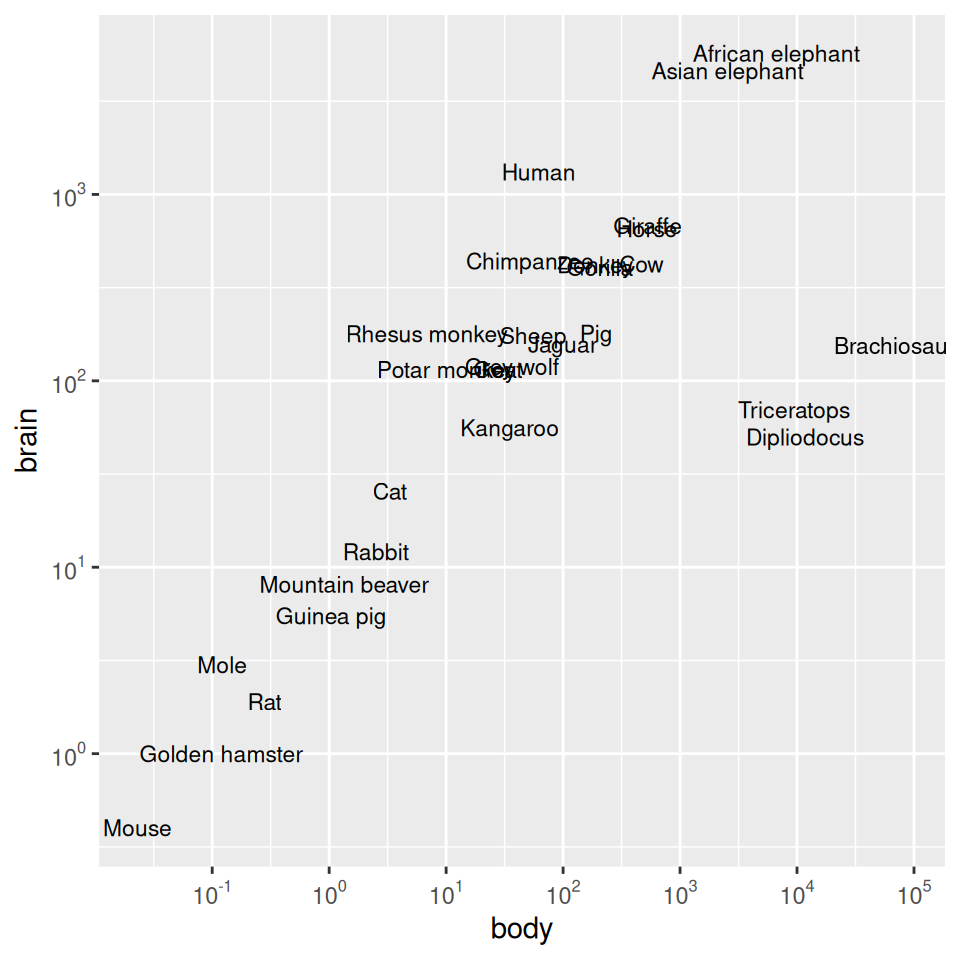Figure 8.27: Scatter plot with log10 x- and y-axes, and with manually specified breaks (left); With exponents for the tick labels (right)

Another way to use log axes is to transform the data before mapping it to the x and y coordinates (Figure 8.28). Technically, the axes are still linear – it’s the quantity that is log-transformed:

``````ggplot(Animals, aes(x = log10(body), y = log10(brain), label = rownames(Animals))) +
geom_text(size = 3)``````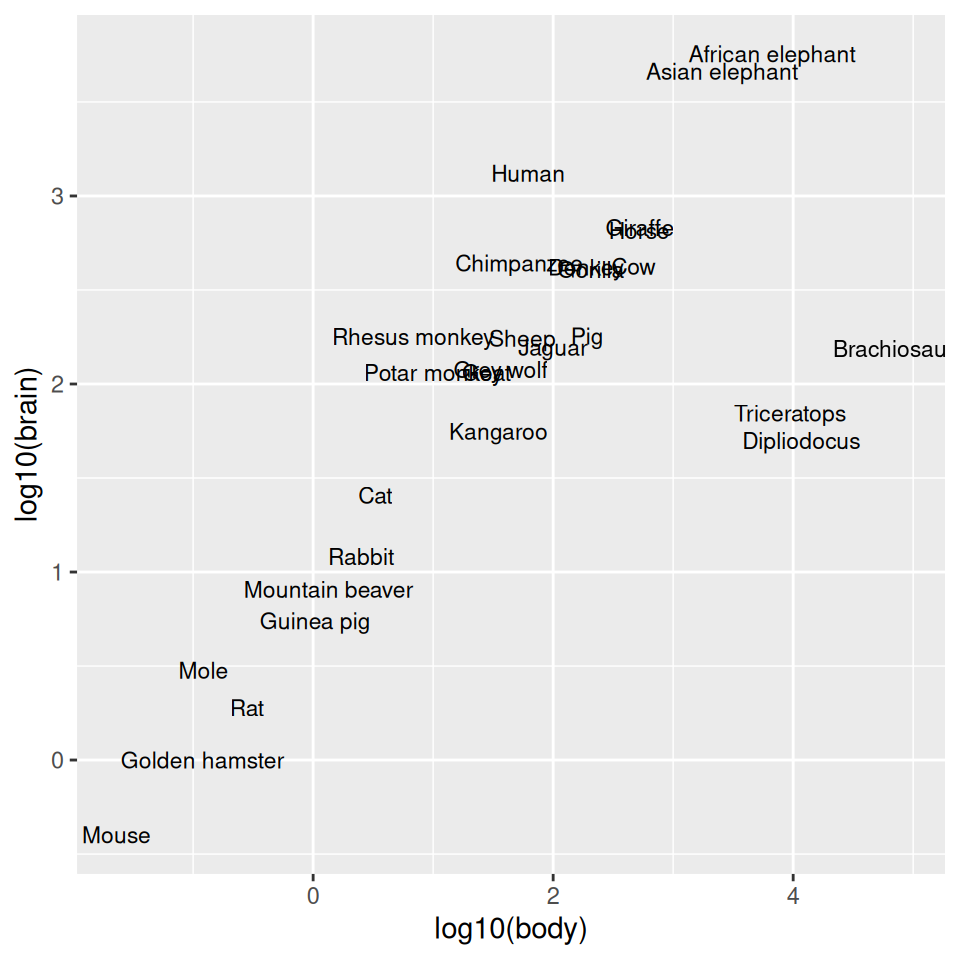Figure 8.28: Plot with log transform before mapping to x- and y-axes

The previous examples used a log10 transformation, but it is possible to use other transformations, such as log2 and natural log, as shown in Figure 8.29. It’s a bit more complicated to use these – `scale_x_log10()` is shorthand, but for these other log scales, we need to spell them out:

``````library(scales)

# Use natural log on x, and log2 on y
animals_plot +
scale_x_continuous(
trans = log_trans(),
breaks = trans_breaks("log", function(x) exp(x)),
labels = trans_format("log", math_format(e^.x))
) +
scale_y_continuous(
trans = log2_trans(),
breaks = trans_breaks("log2", function(x) 2^x),
labels = trans_format("log2", math_format(2^.x))
)``````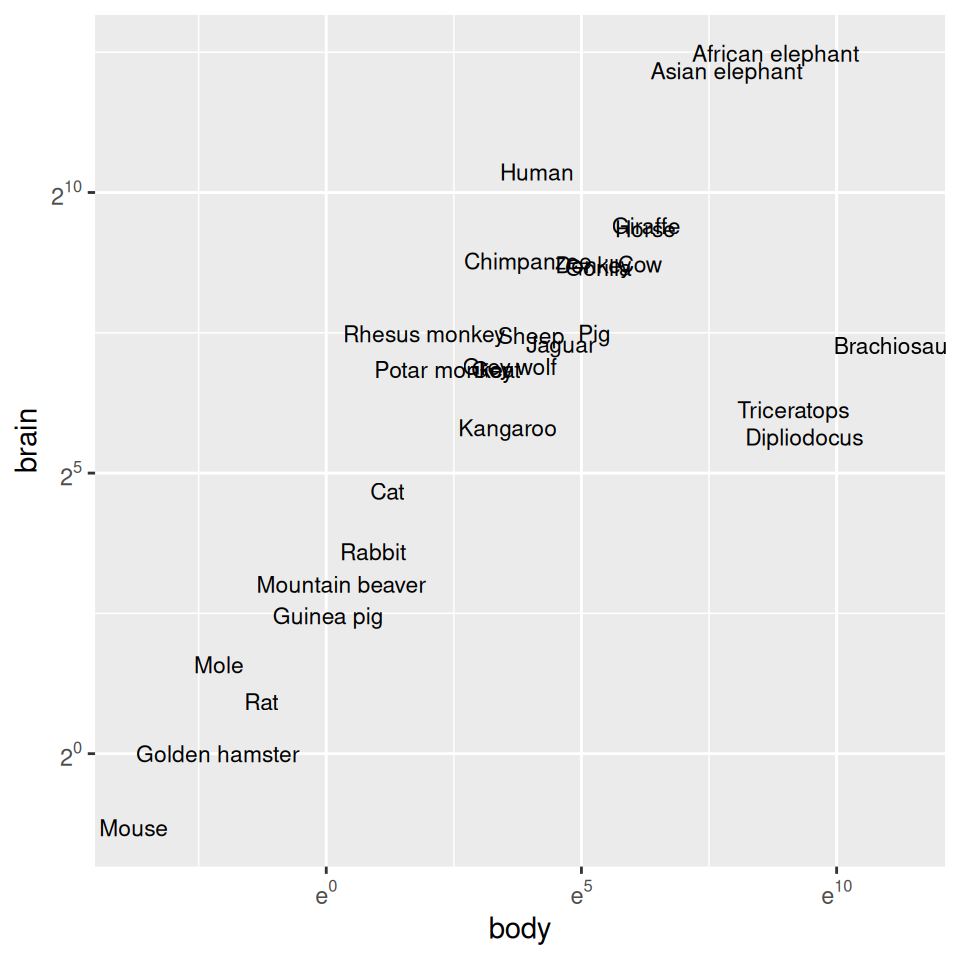Figure 8.29: Plot with exponents in tick labels. Notice that different bases are used for the x and y axes.

It’s possible to use a log axis for just one axis. It is often useful to represent financial data this way, because it better represents proportional change. Figure 8.30 shows Apple’s stock price with linear and log y-axes. The default tick marks might not be spaced well for your graph; they can be set with the breaks in the scale:

``````library(gcookbook)  # Load gcookbook for the aapl data set

ggplot(aapl, aes(x = date,y = adj_price)) +
geom_line()

ggplot(aapl, aes(x = date,y = adj_price)) +
geom_line() +
scale_y_log10(breaks = c(2,10,50,250))``````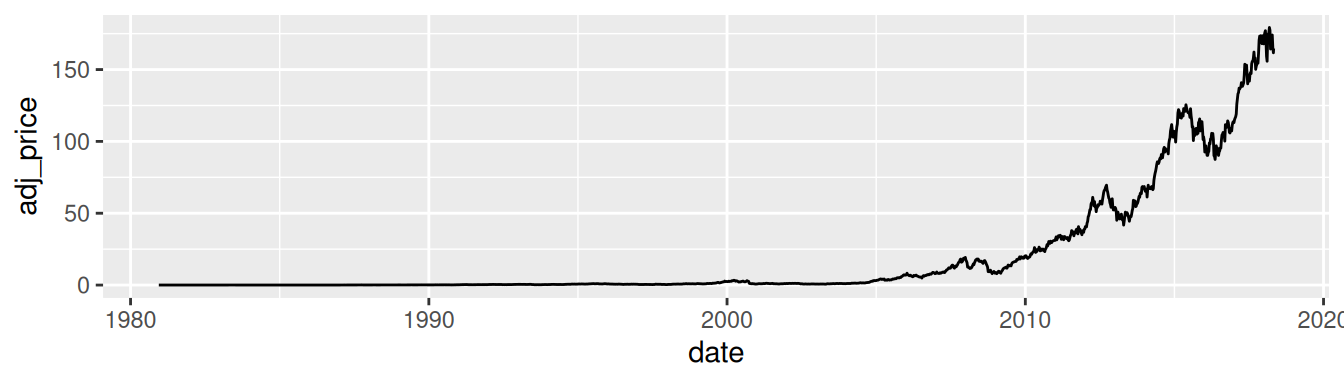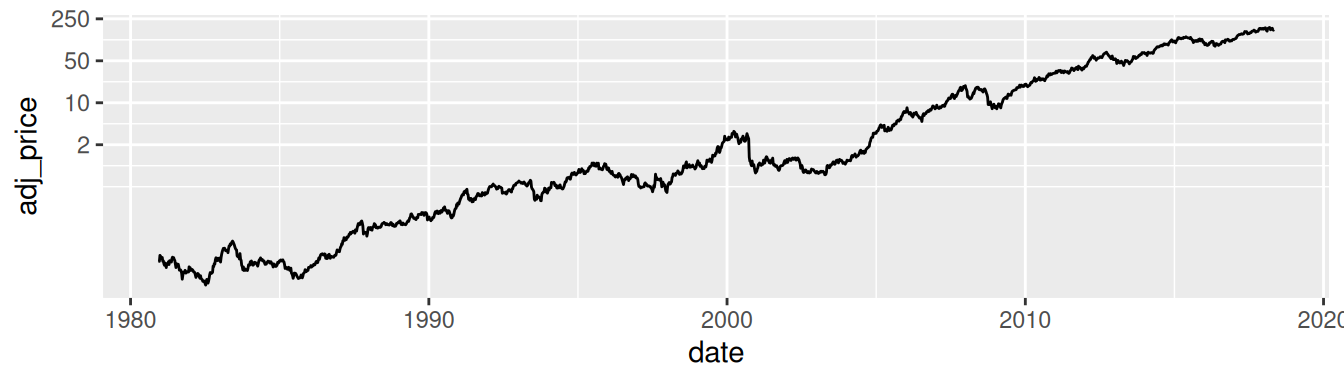Figure 8.30: Top: a stock chart with a linear x-axis and log y-axis; bottom: with manual breaks

## 8.15 Adding Ticks for a Logarithmic Axis

### 8.15.1 Problem

You want to add tick marks with diminishing spacing for a logarithmic axis.

### 8.15.2 Solution

Use `annotation_logticks()` (Figure 8.31):

``````library(MASS)   # Load MASS for the Animals data set
library(scales) # For the trans_format function

# Given a vector x, return a vector of powers of 10 that encompasses all values
# in x.
breaks_log10 <- function(x) {
low <- floor(log10(min(x)))
high <- ceiling(log10(max(x)))

10^(seq.int(low, high))
}

ggplot(Animals, aes(x = body, y = brain, label = rownames(Animals))) +
geom_text(size = 3) +
annotation_logticks() +
scale_x_log10(breaks = breaks_log10,
labels = trans_format(log10, math_format(10^.x))) +
scale_y_log10(breaks = breaks_log10,
labels = trans_format(log10, math_format(10^.x)))``````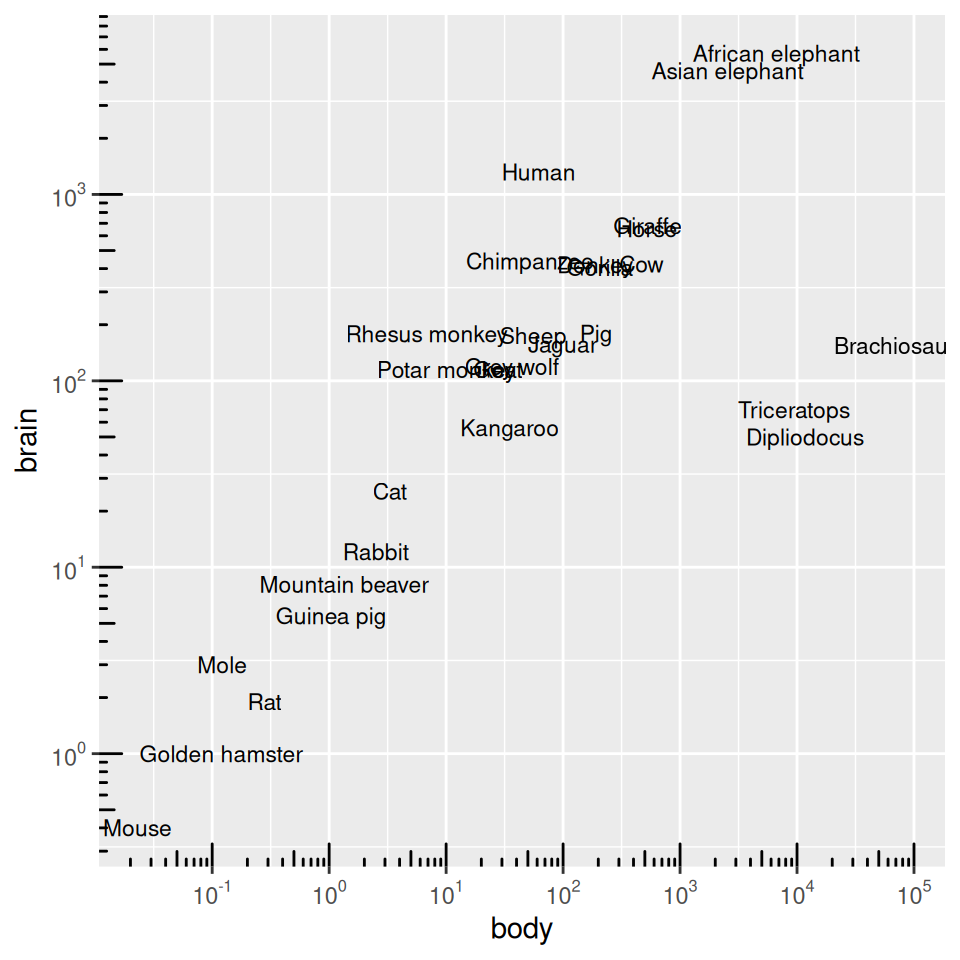Figure 8.31: Log axes with diminishing tick marks

We also defined a function, `breaks_log10()`, which returns all powers of 10 that encompass the range of values passed to it. This tells `scale_x_log10` where to put the breaks. For example:

``````breaks_log10(c(0.12, 6))
#>   0.1  1.0 10.0``````

### 8.15.3 Discussion

The tick marks created by `annotation_logticks()` are actually geoms inside the plotting area. There is a long tick mark at each power of 10, and a mid-length tick mark at each 5.

To get the colors of the tick marks and the grid lines to match up a bit better, you can use `theme_bw()`.

By default, the minor grid lines appear visually halfway between the major grid lines, but this is not the same place as the “5” tick marks on a logarithmic scale. To get them to be the same, we can supply a function for the scales `minor_breaks`.

We’ll define `breaks_5log10()`, which returns 5 times powers of 10 that encompass the values passed to it.

``````breaks_5log10 <- function(x) {
low <- floor(log10(min(x)/5))
high <- ceiling(log10(max(x)/5))

5 * 10^(seq.int(low, high))
}

breaks_5log10(c(0.12, 6))
#>   0.05  0.50  5.00 50.00``````

Then we’ll use that function for the `minor breaks` (Figure 8.32):

``````ggplot(Animals, aes(x = body, y = brain, label = rownames(Animals))) +
geom_text(size = 3) +
annotation_logticks() +
scale_x_log10(breaks = breaks_log10,
minor_breaks = breaks_5log10,
labels = trans_format(log10, math_format(10^.x))) +
scale_y_log10(breaks = breaks_log10,
minor_breaks = breaks_5log10,
labels = trans_format(log10, math_format(10^.x))) +
coord_fixed() +
theme_bw()``````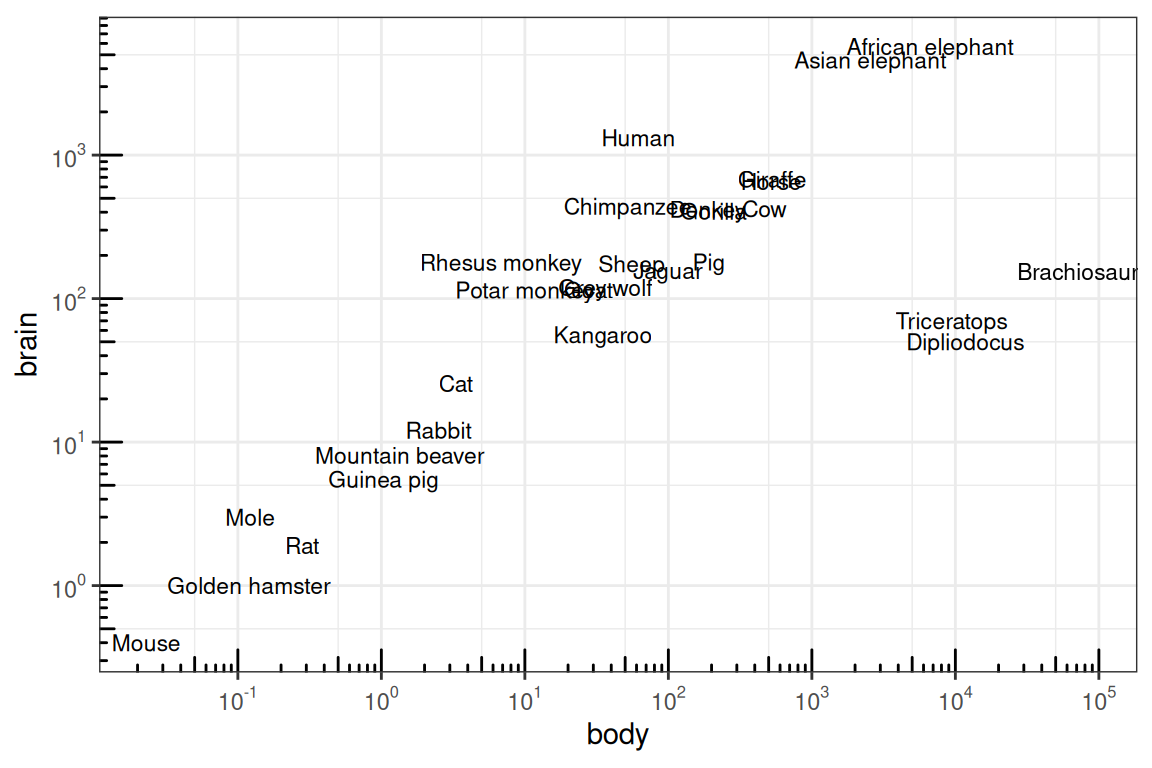Figure 8.32: Log axes with ticks at each 5, and fixed coordinate ratio

## 8.16 Making a Circular Plot

### 8.16.1 Problem

You want to make a circular plot.

### 8.16.2 Solution

Use `coord_polar()`. For this example we’ll use the `wind` data set from gcookbook. It contains samples of wind speed and direction for every 5 minutes throughout a day. The direction of the wind is categorized into 15-degree bins, and the speed is categorized into 5 m/s increments:

``````library(gcookbook)  # Load gcookbook for the wind data set
wind
#>     TimeUTC Temp WindAvg WindMax WindDir SpeedCat DirCat
#> 3         0 3.54    9.52   10.39      89    10-15     90
#> 4         5 3.52    9.10    9.90      92     5-10     90
#> 5        10 3.53    8.73    9.51      92     5-10     90
#>  ...<280 more rows>...
#> 286    2335 6.74   18.98   23.81     250      >20    255
#> 287    2340 6.62   17.68   22.05     252      >20    255
#> 288    2345 6.22   18.54   23.91     259      >20    255``````

We’ll plot a count of the number of samples at each `SpeedCat` and `DirCat` using `geom_histogram()` (Figure 8.33). We’ll set `binwidth` to 15 and make the origin of the histogram start at –7.5, so that each bin is centered around 0, 15, 30, etc.:

``````ggplot(wind, aes(x = DirCat, fill = SpeedCat)) +
geom_histogram(binwidth = 15, boundary = -7.5) +
coord_polar() +
scale_x_continuous(limits = c(0,360))
#> Warning: Removed 8 rows containing missing values (geom_bar).``````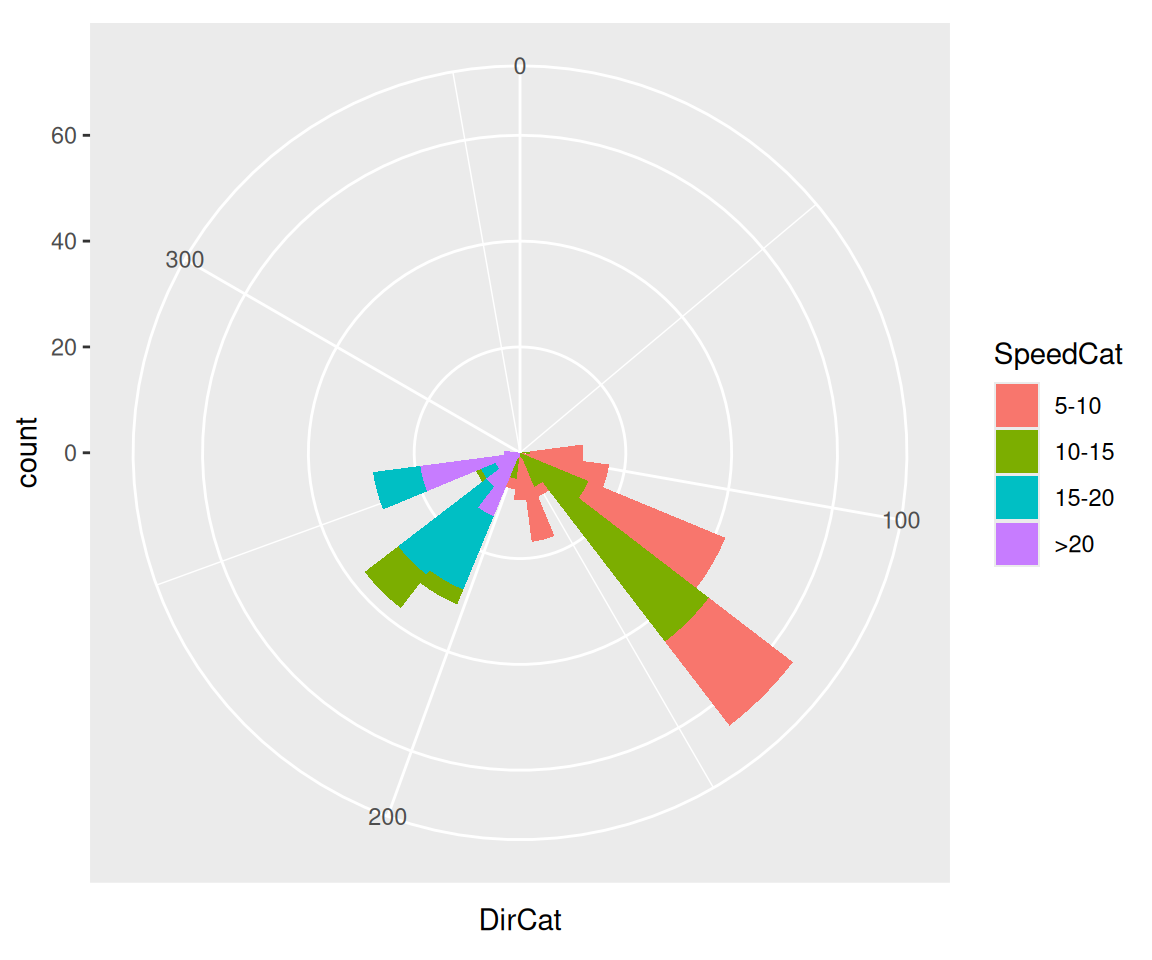Figure 8.33: Polar plot

### 8.16.3 Discussion

Be cautious when using polar plots, since they can perceptually distort the data. In the example here, at 210 degrees there are 15 observations with a speed of 15–20 and 13 observations with a speed of >20, but a quick glance at the picture makes it appear that there are more observations at >20. There are also three observations with a speed of 10–15, but they’re barely visible.

In this example we can make the plot a little prettier by reversing the legend, using a different palette, adding an outline, and setting the breaks to some more familiar numbers (Figure 8.34):

``````ggplot(wind, aes(x = DirCat, fill = SpeedCat)) +
geom_histogram(binwidth = 15, boundary = -7.5, colour = "black", size = .25) +
guides(fill = guide_legend(reverse = TRUE)) +
coord_polar() +
scale_x_continuous(limits = c(0,360),
breaks = seq(0, 360, by = 45),
minor_breaks = seq(0, 360, by = 15)) +
scale_fill_brewer()
#> Warning: Removed 8 rows containing missing values (geom_bar).``````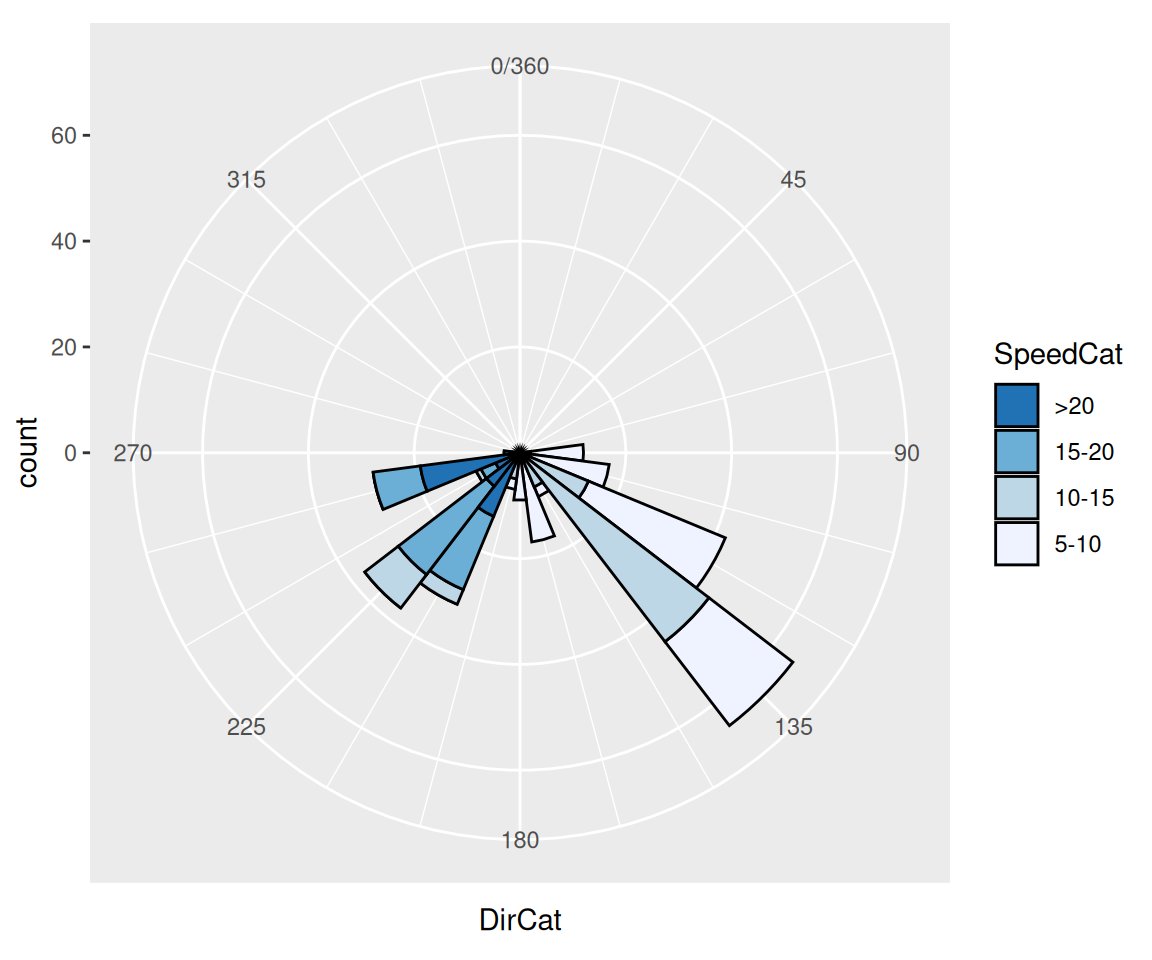Figure 8.34: Polar plot with different colors and breaks

It may also be useful to set the starting angle with the start argument, especially when using a discrete variable for theta. The starting angle is specified in radians, so if you know the adjustment in degrees, you’ll have to convert it to radians:

``coord_polar(start = -45 * pi / 180)``

Polar coordinates can be used with other geoms, including lines and points. There are a few important things to keep in mind when using these geoms. First, by default, for the variable that is mapped to y (or r), the smallest actual value gets mapped to the center; in other words, the smallest data value gets mapped to a visual radius value of 0. You may be expecting a data value of 0 to be mapped to a radius of 0, but to make sure this happens, you’ll need to set the limits.

Next, when using a continuous x (or theta), the smallest and largest data values are merged. Sometimes this is desirable, sometimes not. To change this behavior, you’ll need to set the limits.

Finally, the theta values of the polar coordinates do not wrap around-it is presently not possible to have a geom that crosses over the starting angle (usually vertical).

I’ll illustrate these issues with an example. The following code creates a data frame from the `mdeaths` time series data set and produces the graph shown on the left in Figure 8.35:

``````# Put mdeaths time series data into a data frame
mdeaths_mod <- data.frame(
deaths = as.numeric(mdeaths),
month = as.numeric(cycle(mdeaths))
)

# Calculate average number of deaths in each month
library(dplyr)
mdeaths_mod <- mdeaths_mod %>%
group_by(month) %>%
summarise(deaths = mean(deaths))

mdeaths_mod
#> # A tibble: 12 x 2
#>   month   deaths
#>   <dbl>  <dbl>
#> 1     1 2129.833
#> 2     2 2081.333
#> 3     3 1970.500
#> 4     4 1657.333
#> 5     5 1314.167
#> 6     6 1186.833
#> 7     7 1136.667
#> 8     8 1037.667
#> ... with 4 more rows

# Create the base plot
mdeaths_plot <- ggplot(mdeaths_mod, aes(x = month, y = deaths)) +
geom_line() +
scale_x_continuous(breaks = 1:12)

# With coord_polar
mdeaths_plot + coord_polar()``````

The first problem is that the data values (ranging from about 1000 to 2100) are mapped to the radius such that the smallest data value is at radius 0. We’ll fix this by setting the y (or r) limits from 0 to the maximum data value, as shown in the graph on the right in Figure 8.35:

``````# With coord_polar and y (r) limits going to zero
mdeaths_plot +
coord_polar() +
ylim(0, max(mdeaths_mod\$deaths))``````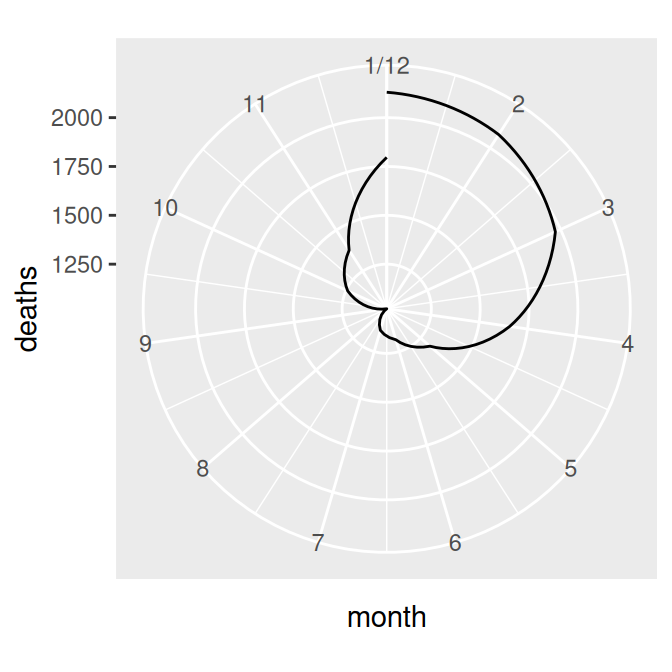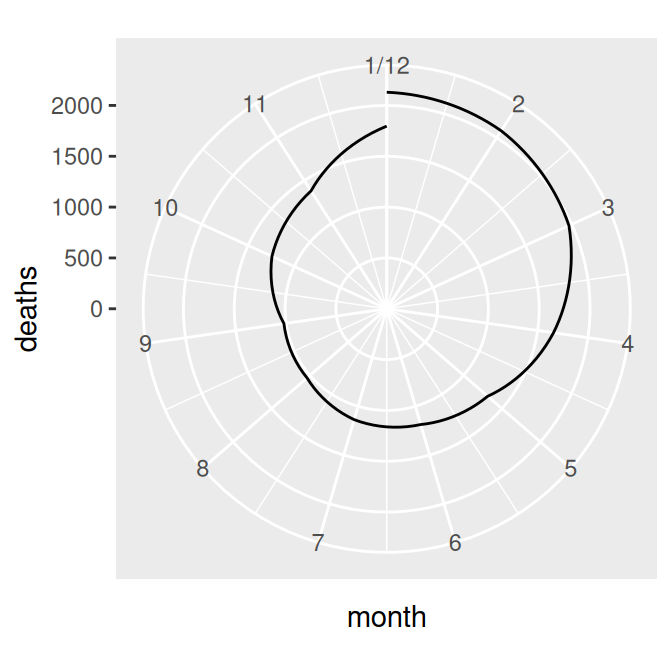Figure 8.35: Polar plot with line (notice the data range of the radius) (left); With the radius representing a data range starting from zero (right)

The next problem is that the lowest and highest month values, 1 and 12, are shown at the same angle. We’ll fix this by setting the x limits from 0 to 12, creating the graph on the left in Figure 8.36 (notice that using `xlim()` overrides the `scale_x_continuous()` in `p`, so it no longer displays breaks for each month; see Recipe 8.2 for more information):

``````mdeaths_plot +
coord_polar() +
ylim(0, max(mdeaths_mod\$deaths)) +
xlim(0, 12)``````

There’s one last issue, which is that the beginning and end aren’t connected. To fix that, we need to modify our data frame by adding one row with a month of 0 that has the same value as the row with month 12. This will make the starting and ending points the same, as in the graph on the right in Figure 8.36 (alternatively, we could add a row with month 13, instead of month 0):

``````# Connect the lines by adding a value for 0 that is the same as 12
mdeaths_x <- mdeaths_mod[mdeaths_mod\$month==12, ]
mdeaths_x\$month <- 0
mdeaths_new <- rbind(mdeaths_x, mdeaths_mod)

# Make the same plot as before, but with the new data, by using %+%
mdeaths_plot %+%
mdeaths_new +
coord_polar() +
ylim(0, max(mdeaths_mod\$deaths))``````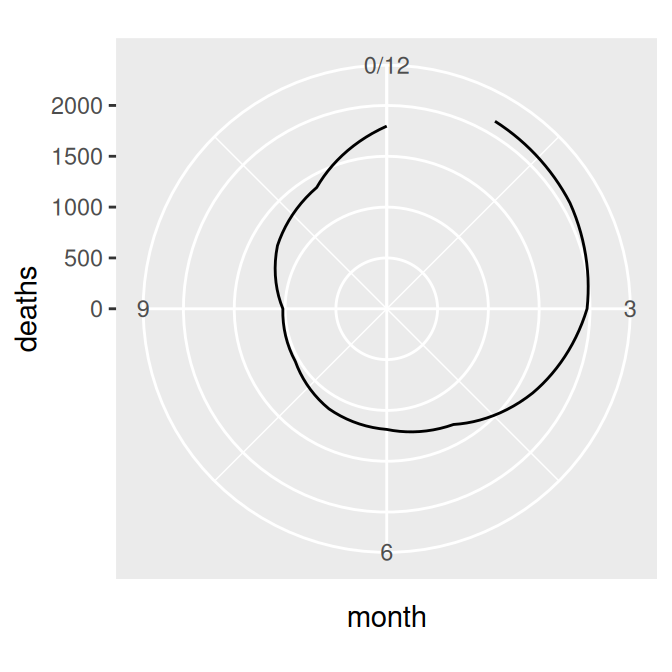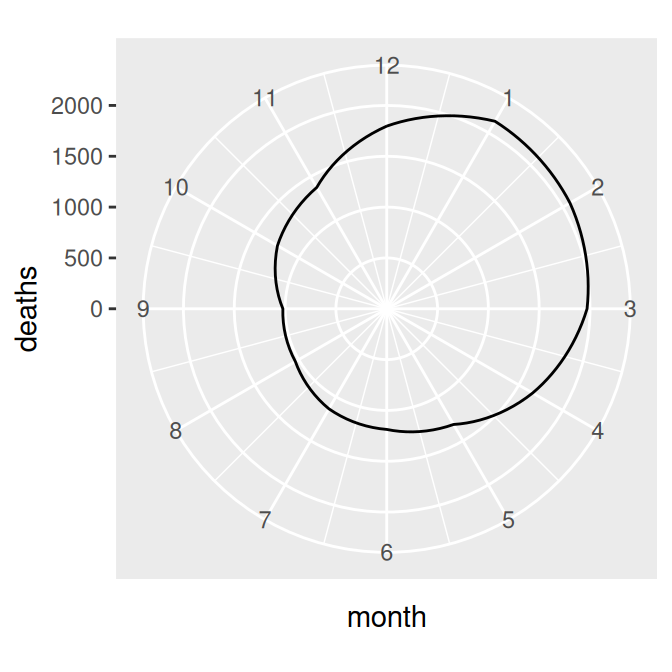Figure 8.36: Polar plot with theta representing x values from 0 to 12 (left); The gap is filled in by adding a dummy data point for month 0 (right)

Note

Notice the use of the `%+%` operator. When you add a data frame to a ggplot object with `%+%`, it replaces the default data frame in the ggplot object. In this case, it changed the default data frame for `p` from `md` to `mdnew`.

See Recipe 10.4 for more about reversing the direction of a legend.

See Recipe 8.6 for more about specifying which values will have tick marks (breaks) and labels.

## 8.17 Using Dates on an Axis

### 8.17.1 Problem

You want to use dates on an axis.

### 8.17.2 Solution

Map a column of class `Date` to the x- or y-axis. We’ll use the `economics` data set for this example:

``````economics
#> # A tibble: 574 x 6
#>   date         pce    pop psavert uempmed unemploy
#>   <date>     <dbl>  <dbl>   <dbl>   <dbl>    <dbl>
#> 1 1967-07-01  507. 198712    12.6     4.5     2944
#> 2 1967-08-01  510. 198911    12.6     4.7     2945
#> 3 1967-09-01  516. 199113    11.9     4.6     2958
#> 4 1967-10-01  512. 199311    12.9     4.9     3143
#> 5 1967-11-01  517. 199498    12.8     4.7     3066
#> 6 1967-12-01  525. 199657    11.8     4.8     3018
#> # … with 568 more rows``````

The column date is an object of class `Date`, and mapping it to x will produce the result shown in Figure 8.37:

``````ggplot(economics, aes(x = date, y = psavert)) +
geom_line()``````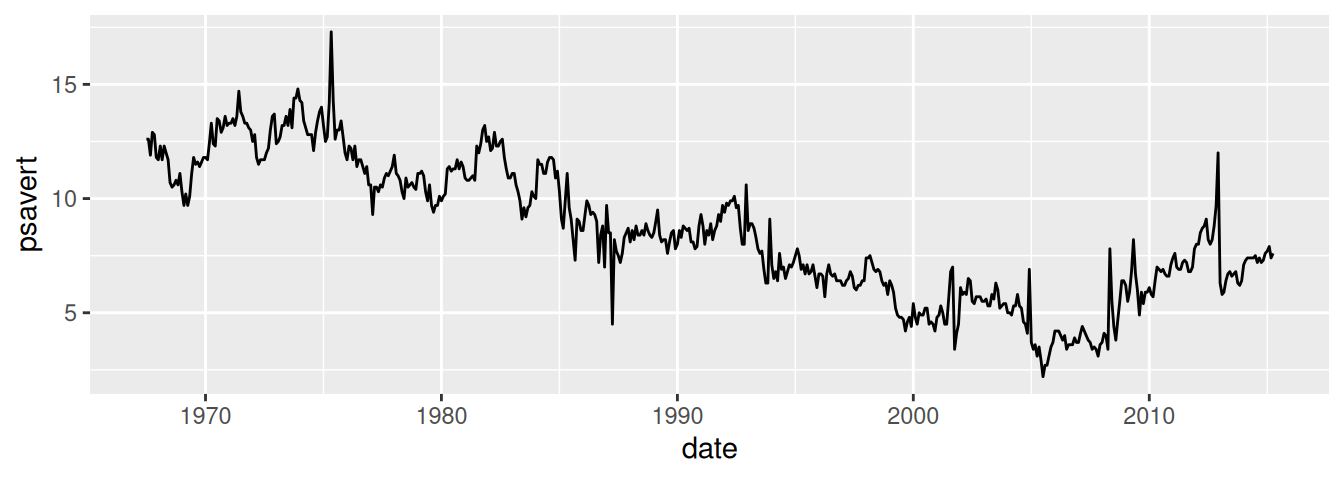Figure 8.37: Dates on the x-axis

### 8.17.3 Discussion

ggplot handles two kinds of time-related objects: dates (objects of class `Date`) and date-times (objects of class `POSIXt`). The difference between these is that `Date` objects represent dates and have a resolution of one day, while `POSIXt` objects represent moments in time and have a resolution of a fraction of a second.

Specifying the breaks is similar to with a numeric axis – the main difference is in specifying the sequence of dates to use. We’ll use a subset of the `economics` data, ranging from mid-1992 to mid-1993. If breaks aren’t specified, they will be automatically selected, as shown in Figure 8.38 (top):

``````library(dplyr)

# Take a subset of economics
econ_mod <- economics %>%
filter(date >= as.Date("1992-05-01") & date <  as.Date("1993-06-01"))

# Create the base plot, which does not specify the breaks
econ_plot <- ggplot(econ_mod, aes(x = date, y = psavert)) +
geom_line()

econ_plot``````

The breaks can be created by using the `seq()` function with starting and ending dates, and an interval (Figure 8.38, bottom):

``````# Specify breaks as a Date vector
datebreaks <- seq(as.Date("1992-06-01"), as.Date("1993-06-01"), by = "2 month")

# Use breaks, and rotate text labels
econ_plot +
scale_x_date(breaks = datebreaks) +
theme(axis.text.x = element_text(angle = 30, hjust = 1))``````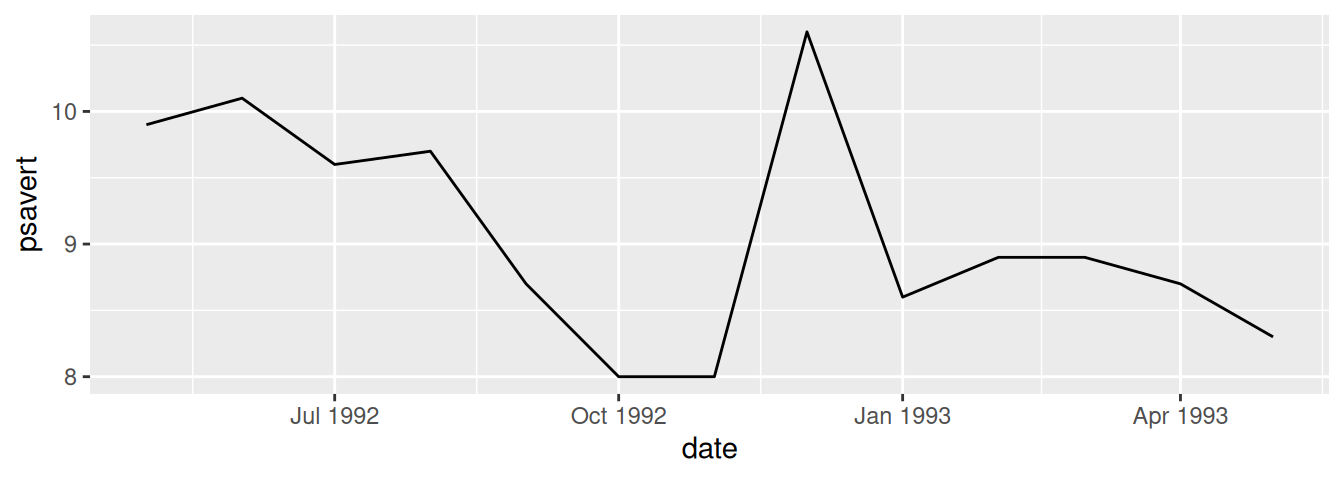Figure 8.38: Top: with default breaks on the x-axis; bottom: with breaks specified

Notice that the formatting of the breaks changed. You can specify the formatting by using the `date_format()` function from the scales package. Here we’ll use `"%Y %b"`, which results in a format like `"1992 Jun"`, as shown in Figure 8.39:

``````library(scales)

econ_plot +
scale_x_date(breaks = datebreaks, labels = date_format("%Y %b")) +
theme(axis.text.x = element_text(angle = 30, hjust = 1))``````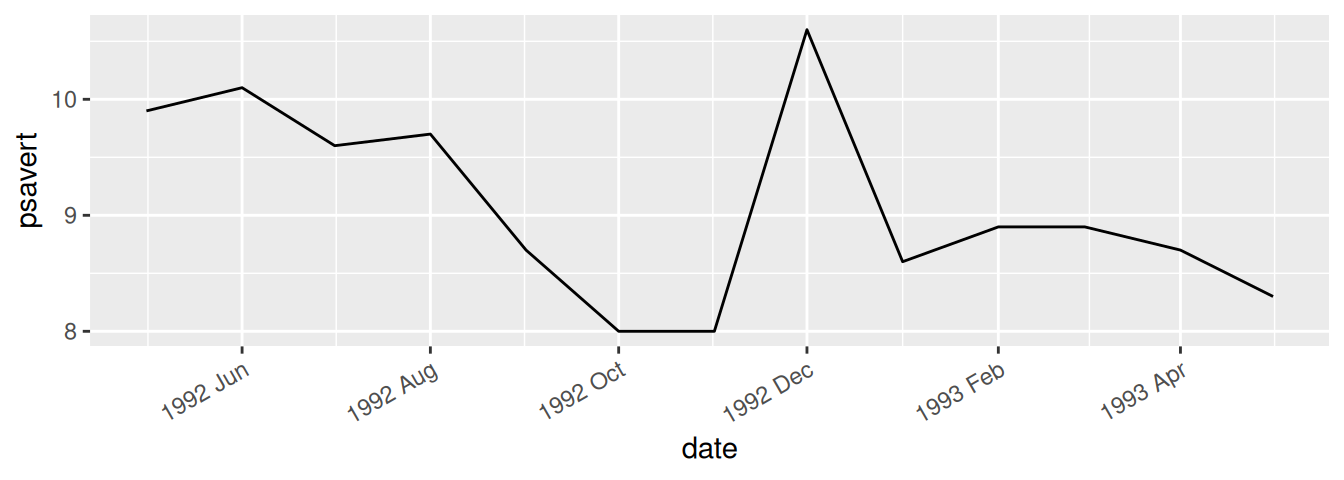Figure 8.39: Line graph with date format specified

Common date format options are shown in Table 8.1. They are to be put in a string that is passed to `date_format()`, and the format specifiers will be replaced with the appropriate values. For example, if you use `"%B %d, %Y"`, it will result in labels like “June 01, 1992”.

Table 8.1: Date format options
Option Description
`%Y` Year with century (2012)
`%y` Year without century (12)
`%m` Month as a decimal number (08)
`%b` Abbreviated month name in current locale (Aug)
`%B` Full month name in current locale (August)
`%d` Day of month as a decimal number (04)
`%U` Week of the year as a decimal number, with Sunday as the first day of the week (00–53)
`%W` Week of the year as a decimal number, with Monday as the first day of the week (00–53)
`%w` Day of week (0–6, Sunday is 0)
`%a` Abbreviated weekday name (Thu)
`%A` Full weekday name (Thursday)

Some of these items are specific to the computer’s locale. Months and days have different names in different languages (the examples here are generated with a US locale). You can change the locale with `Sys.setlocale()`. For example, this will change the date formatting to use an Italian locale:

``````# Mac and Linux
Sys.setlocale("LC_TIME", "it_IT.UTF-8")

# Windows
Sys.setlocale("LC_TIME", "italian")``````

Note that the locale names may differ between platforms, and your computer must have support for the locale installed at the operating system level.

See `?Sys.setlocale` for more about setting the locale.

See `?strptime` for information about converting strings to dates, and for information about formatting the date output.

## 8.18 Using Relative Times on an Axis

### 8.18.1 Problem

You want to use relative times on an axis.

### 8.18.2 Solution

Times are commonly stored as numbers. For example, the time of day can be stored as a number representing the hour. Time can also be stored as a number representing the number of minutes or seconds from some starting time. In these cases, you map a value to the x- or y-axis and use a formatter to generate the appropriate axis labels (Figure 8.40):

``````# Convert WWWusage time-series object to data frame
www <- data.frame(
minute = as.numeric(time(WWWusage)),
users  = as.numeric(WWWusage)
)

# Define a formatter function - converts time in minutes to a string
timeHM_formatter <- function(x) {
h <- floor(x/60)
m <- floor(x %% 60)
lab <- sprintf("%d:%02d", h, m) # Format the strings as HH:MM
return(lab)
}

# Default x axis
ggplot(www, aes(x = minute, y = users)) +
geom_line()

# With formatted times
ggplot(www, aes(x = minute, y = users)) +
geom_line() +
scale_x_continuous(
name = "time",
breaks = seq(0, 100, by = 10),
labels = timeHM_formatter
)``````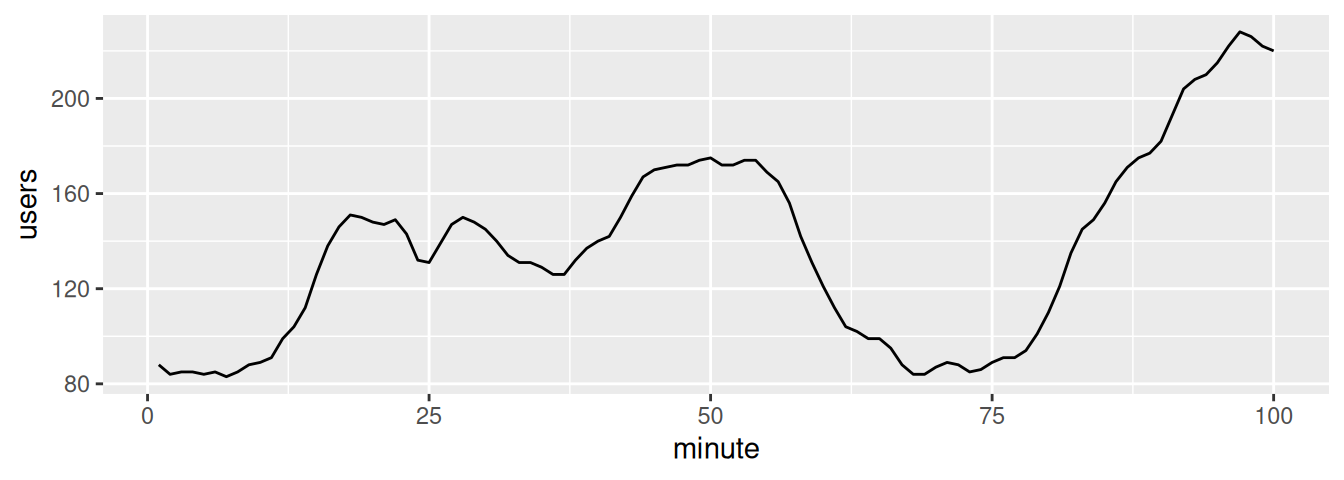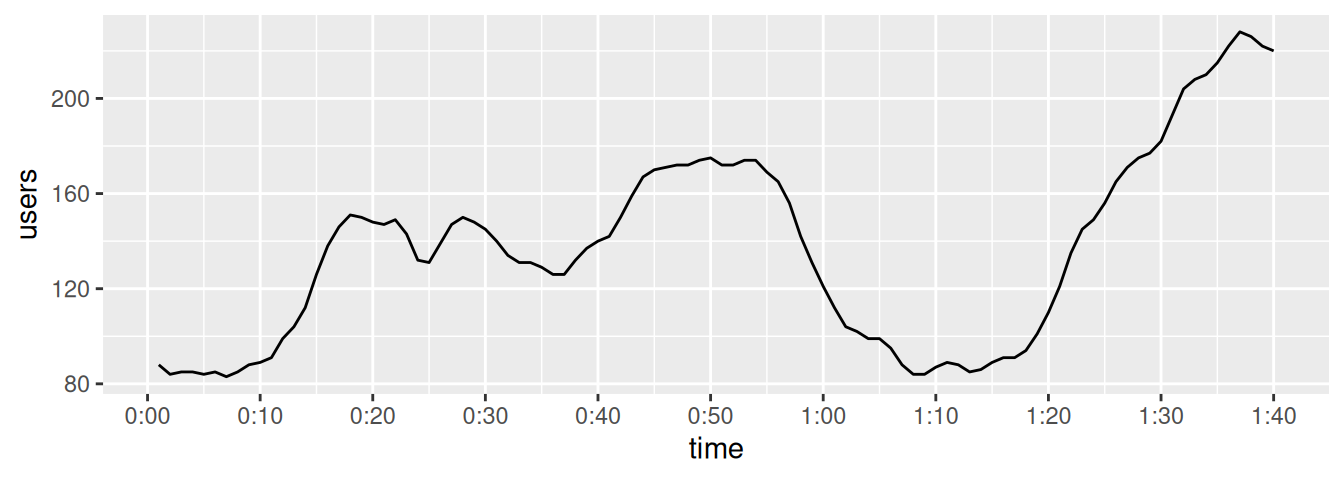Figure 8.40: Top: relative times on x-axis; bottom: with formatted times

### 8.18.3 Discussion

In some cases it might be simpler to specify the breaks and labels manually, with something like this:

``````scale_x_continuous(
breaks = c(0, 20, 40, 60, 80, 100),
labels = c("0:00", "0:20", "0:40", "1:00", "1:20", "1:40")
)``````

In the preceding example, we used the `timeHM_formatter()` function to convert the numeric time (in minutes) to a string like `"1:10"`:

``````timeHM_formatter(c(0, 50, 51, 59, 60, 130, 604))
#>  "0:00"  "0:50"  "0:51"  "0:59"  "1:00"  "2:10"  "10:04"``````

To convert to HH:MM:SS format, you can use the following formatter function:

``````timeHMS_formatter <- function(x) {
h <- floor(x/3600)
m <- floor((x/60) %% 60)
s <- round(x %% 60)                       # Round to nearest second
lab <- sprintf("%02d:%02d:%02d", h, m, s) # Format the strings as HH:MM:SS
lab <- sub("^00:", "", lab)               # Remove leading 00: if present
lab <- sub("^0", "", lab)                 # Remove leading 0 if present
return(lab)
}``````

Running it on some sample numbers yields:

``````timeHMS_formatter(c(20, 3000, 3075, 3559.2, 3600, 3606, 7813.8))
#>  "0:20"    "50:00"   "51:15"   "59:19"   "1:00:00" "1:00:06" "2:10:14"``````